# ML Aggarwal Solutions For Class 10 Maths Chapter 11 Section Formula

ML Aggarwal Solutions For Class 10 Maths Chapter 11 Section Formula provides students an advantage with practical questions. The clear diagrams given in our solutions help students better understand the concept. This chapter deals with coordinates of the midpoint of a line segment, section formula and distance formula. BYJU’S provides precise solutions prepared by our experts. ML Aggarwal Solutions For Class 10 can be downloaded in PDF format for free.

This chapter explains the different applications of section formula.

ML Aggarwal Solutions For Class 10 help students not only to strengthen their foundation in the subject, but also to crack different types of problems easily. Visit BYJU’S to get more information on concepts and a greater level of understanding Section Formula and its solutions.

## ML Aggarwal Solutions for Class 10 Maths Chapter 11 :-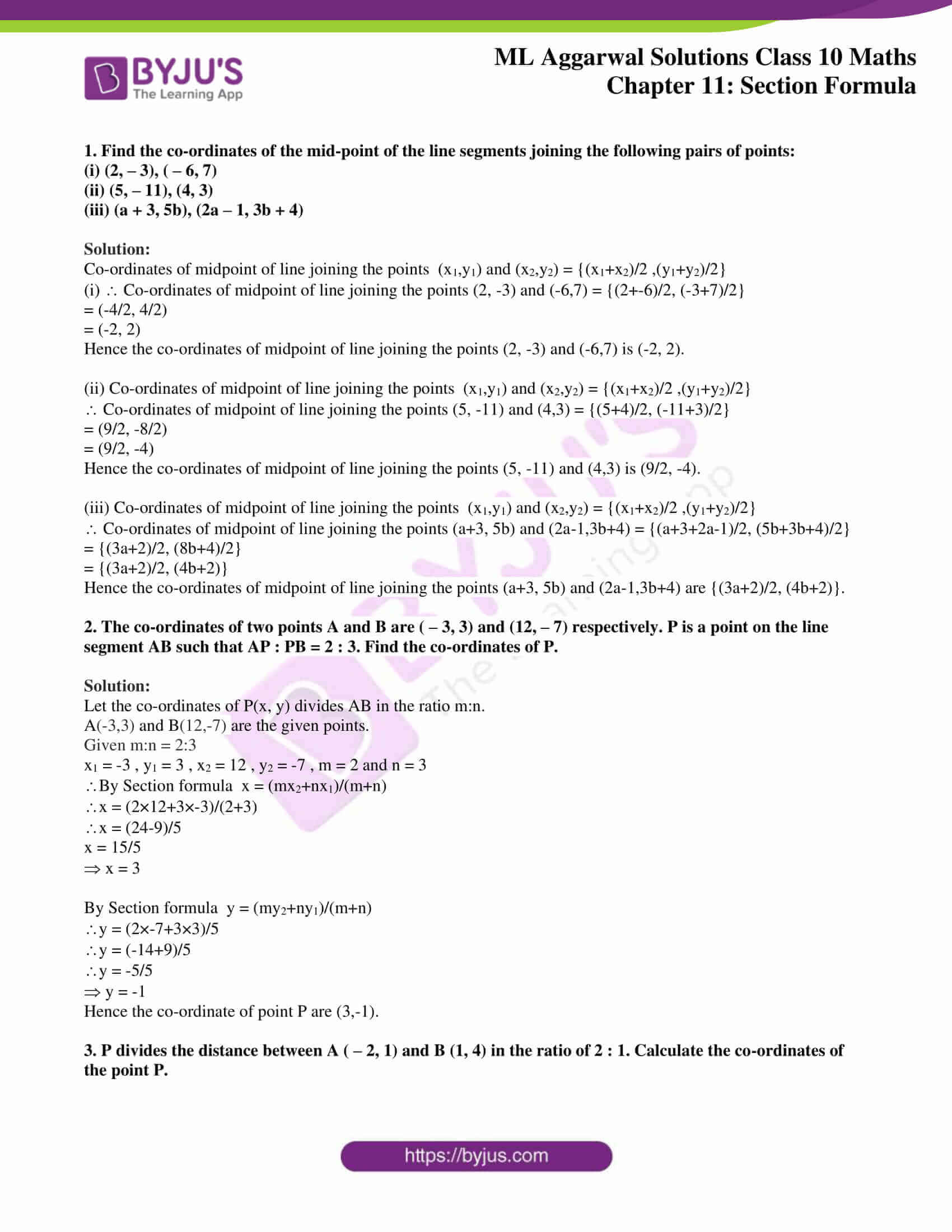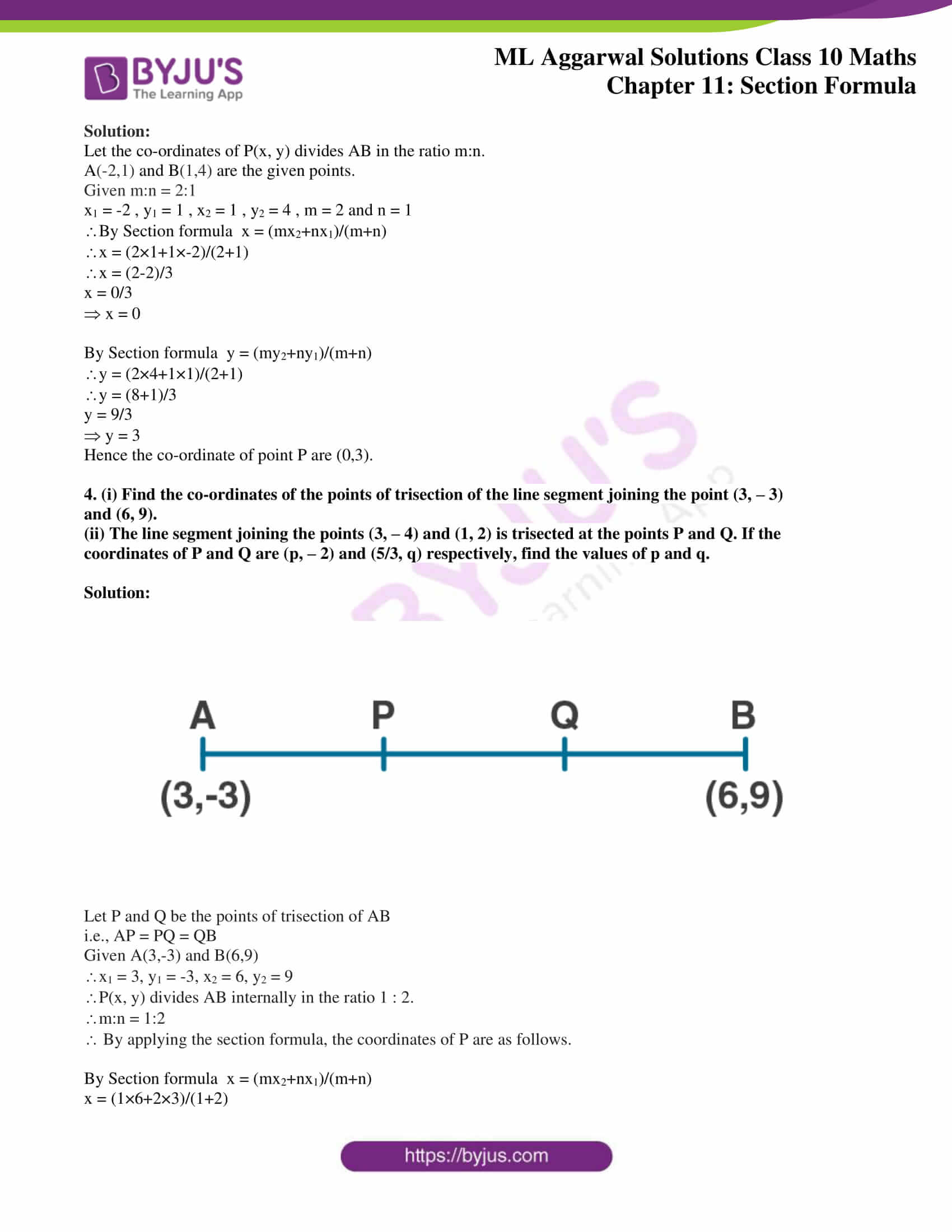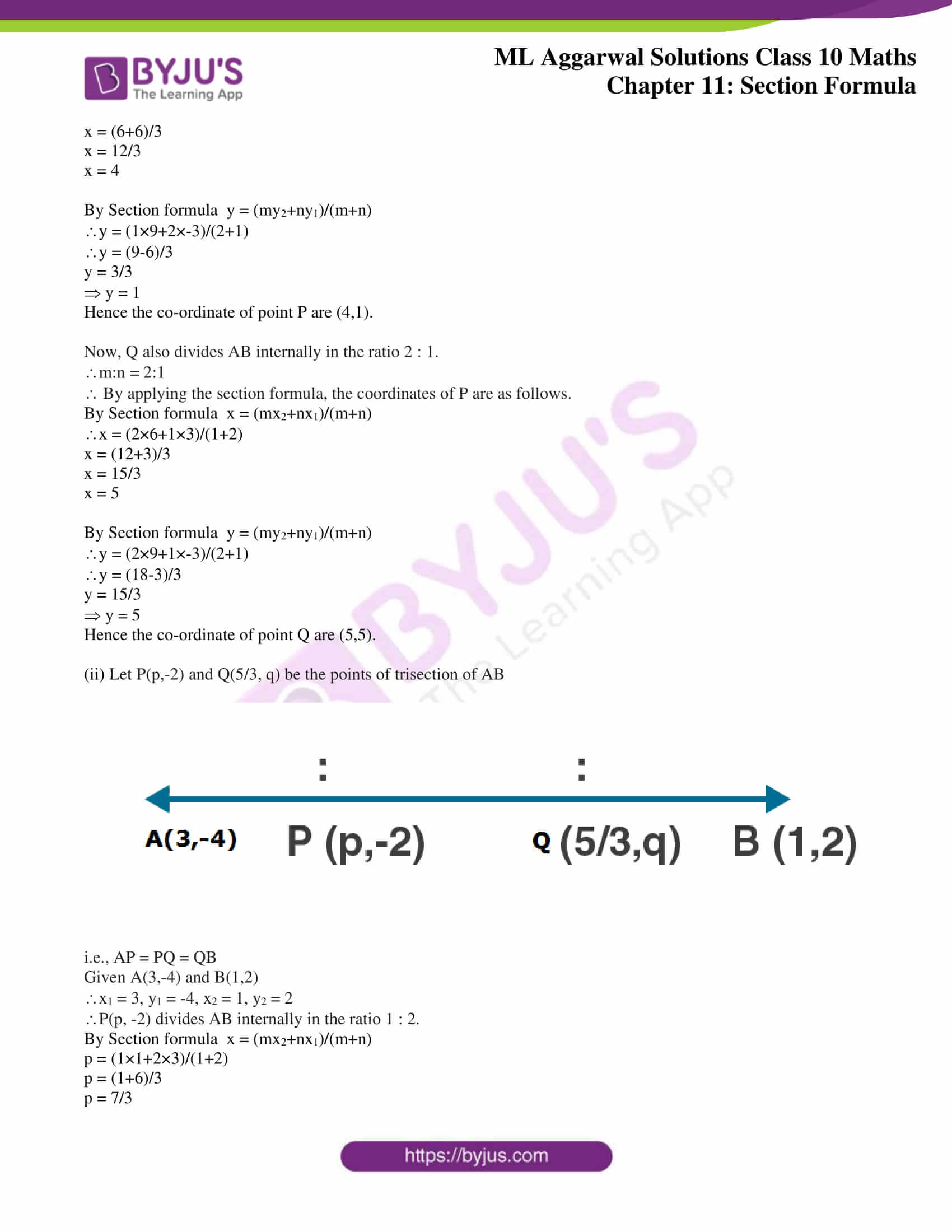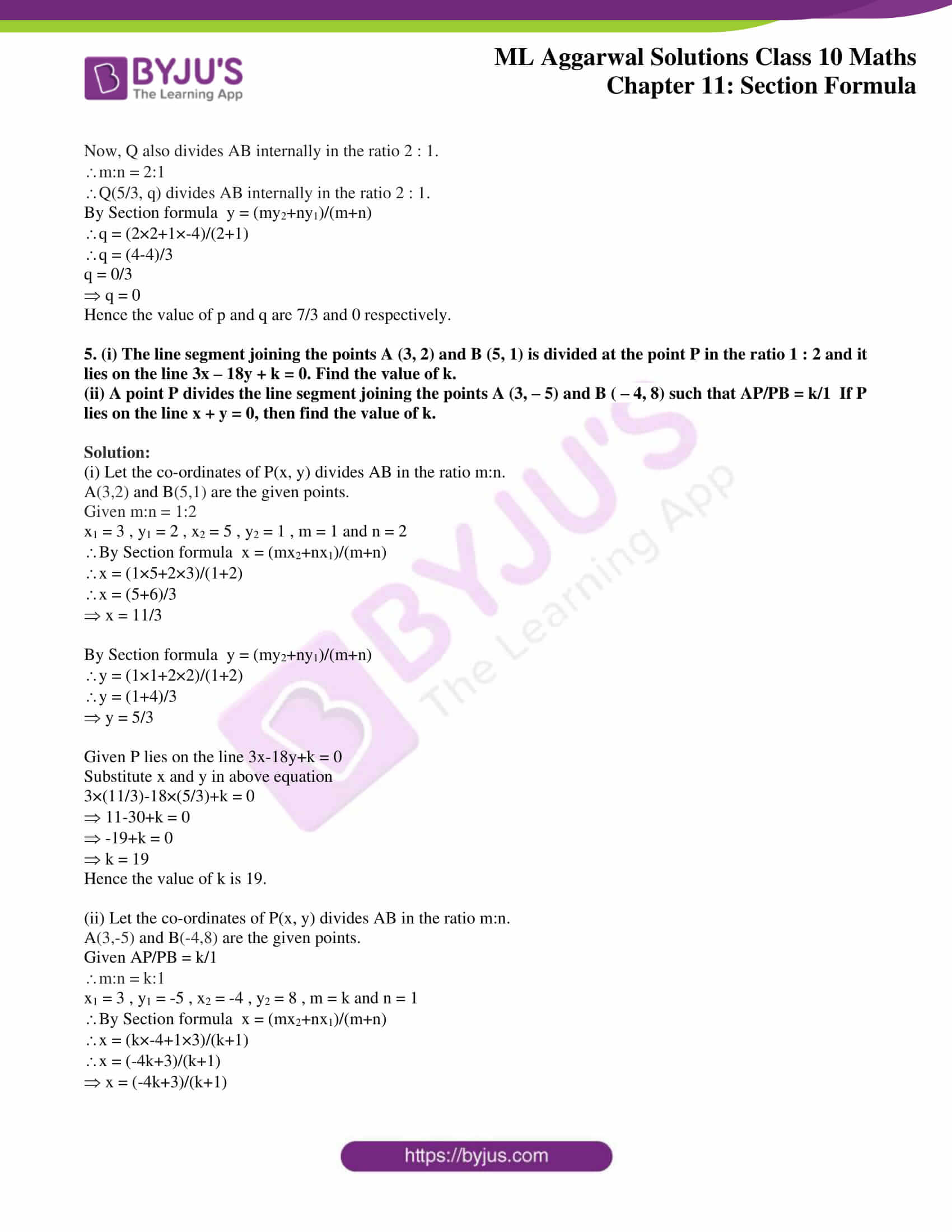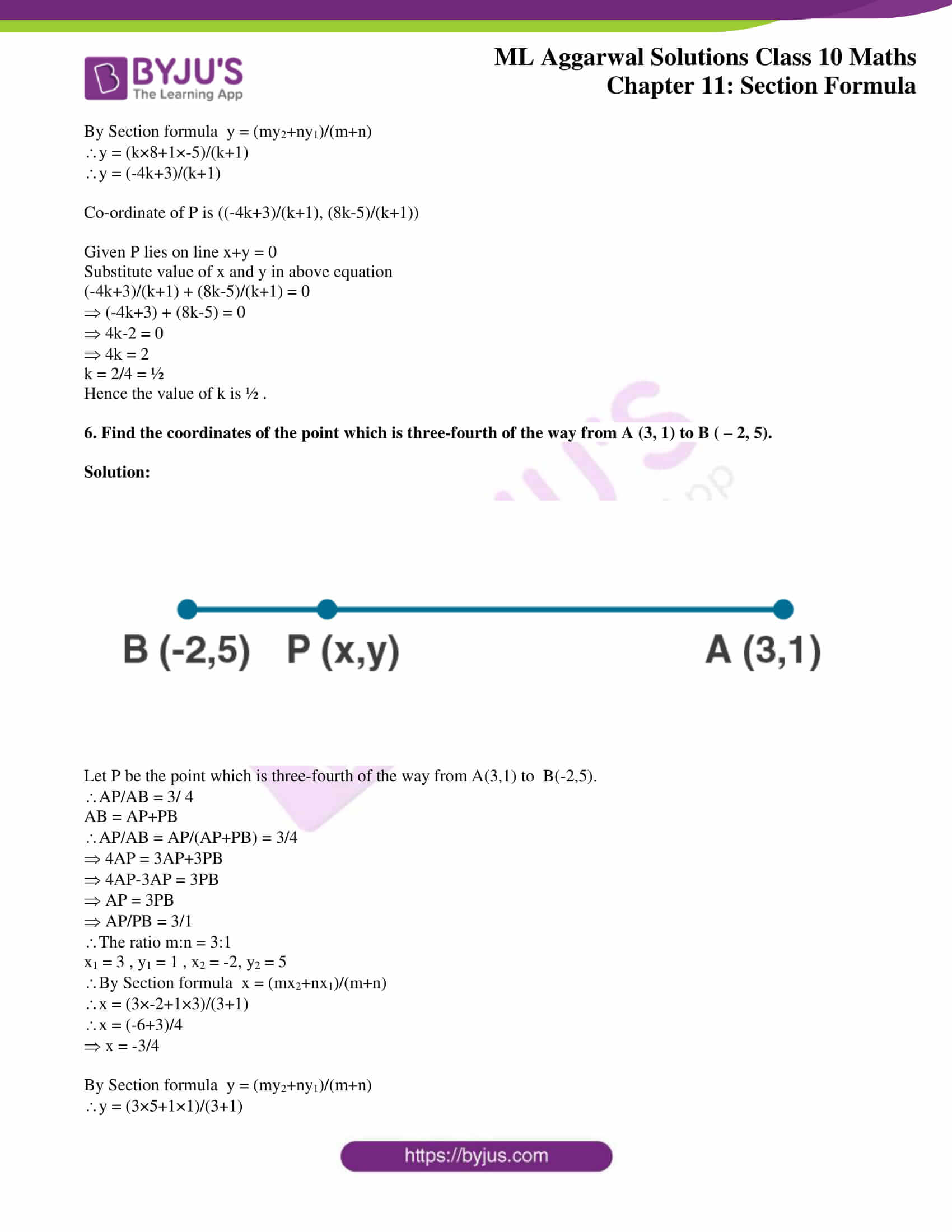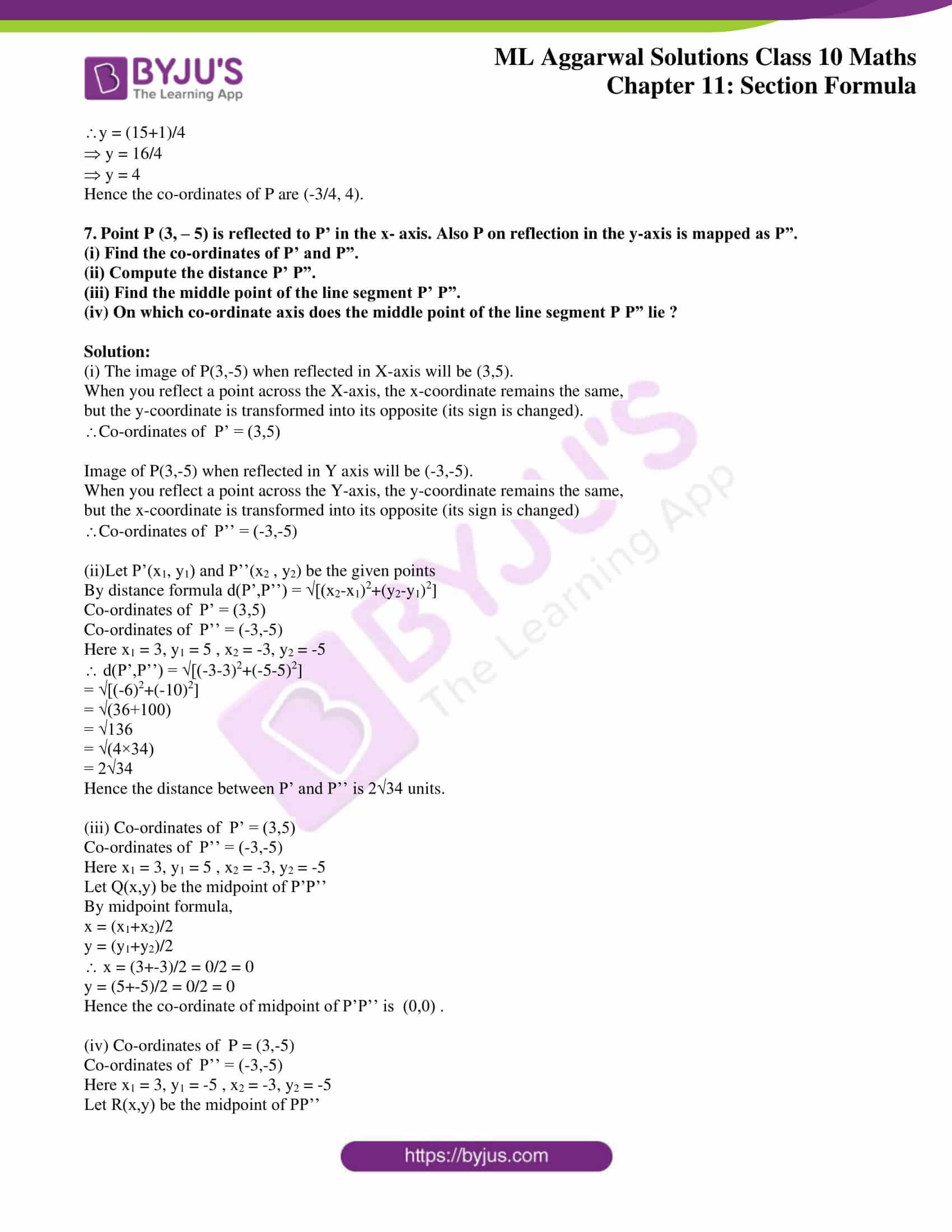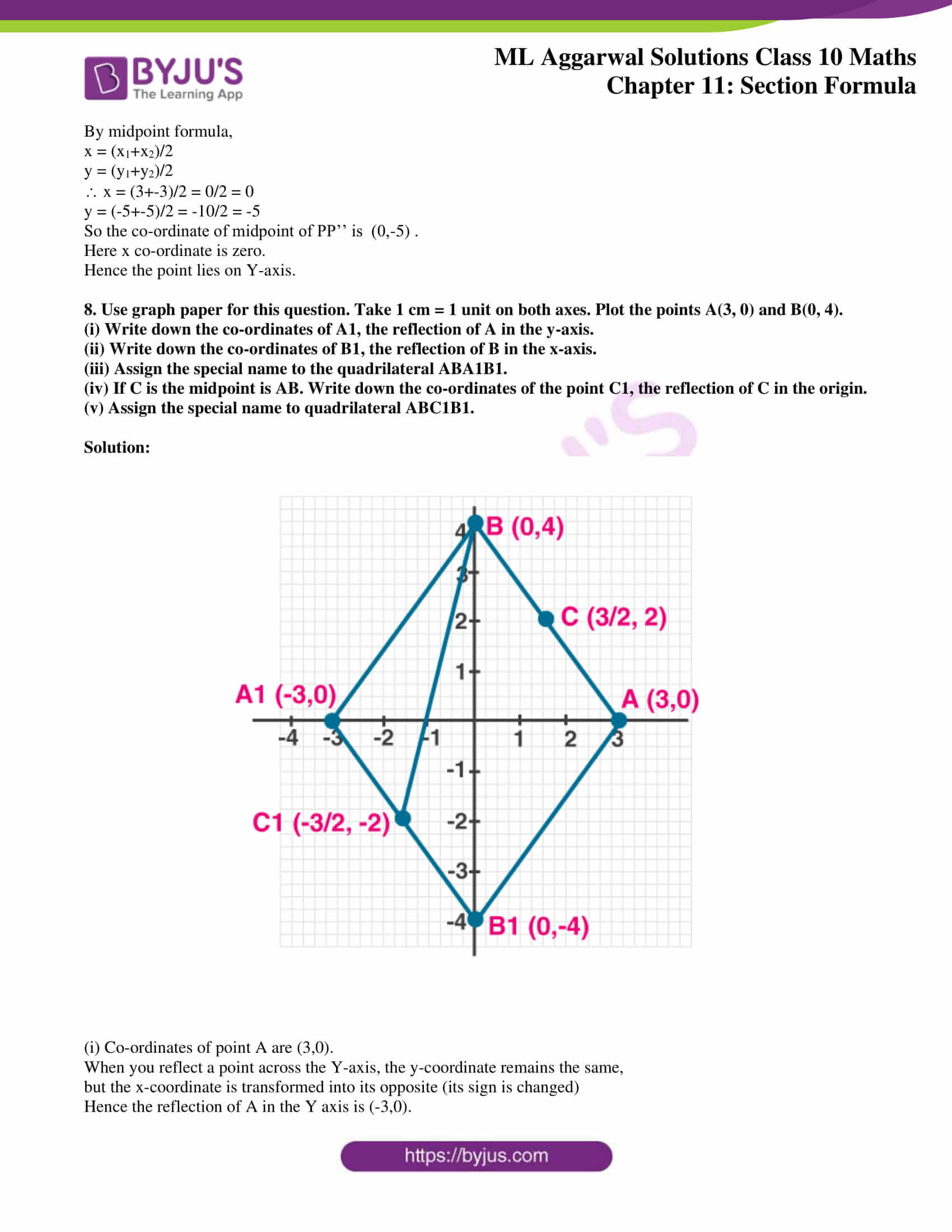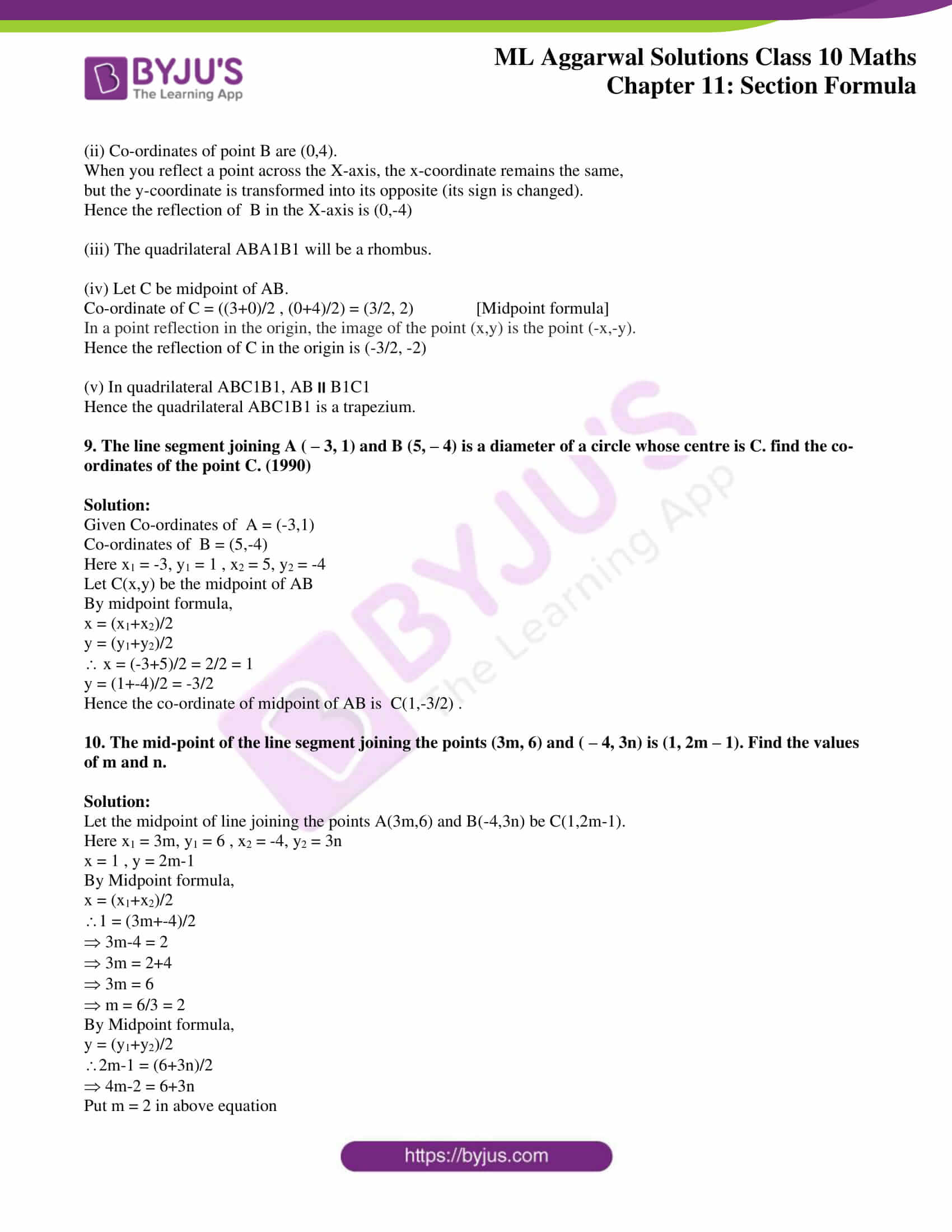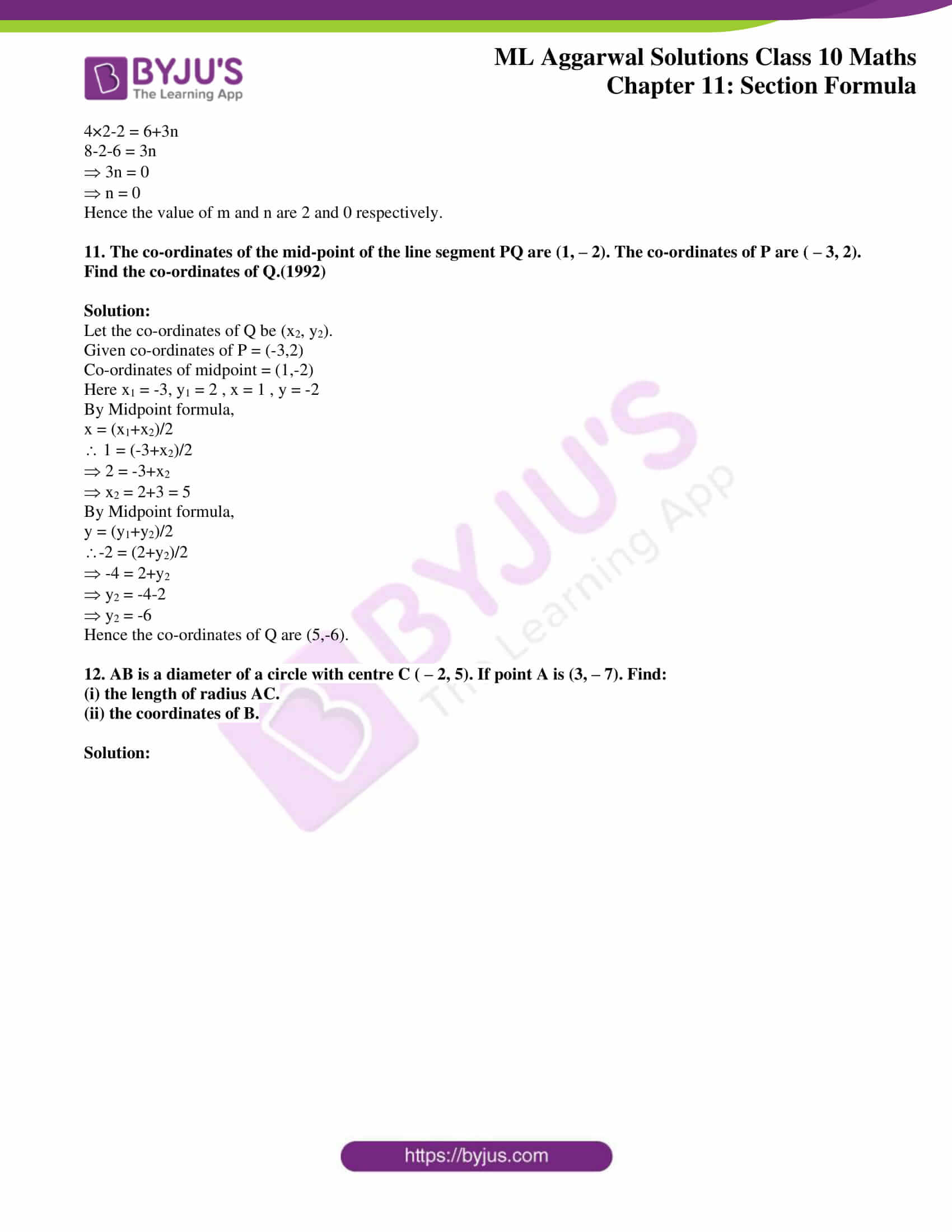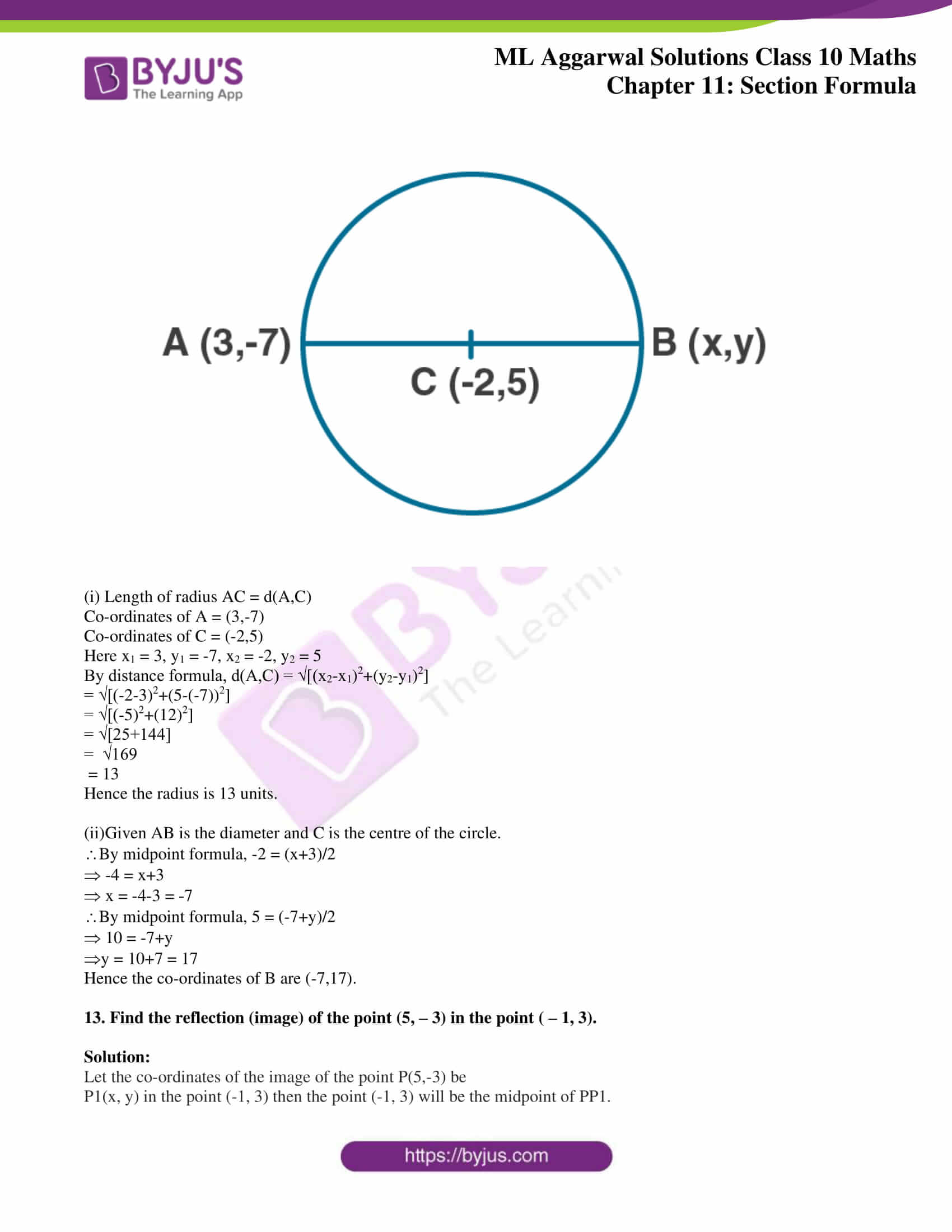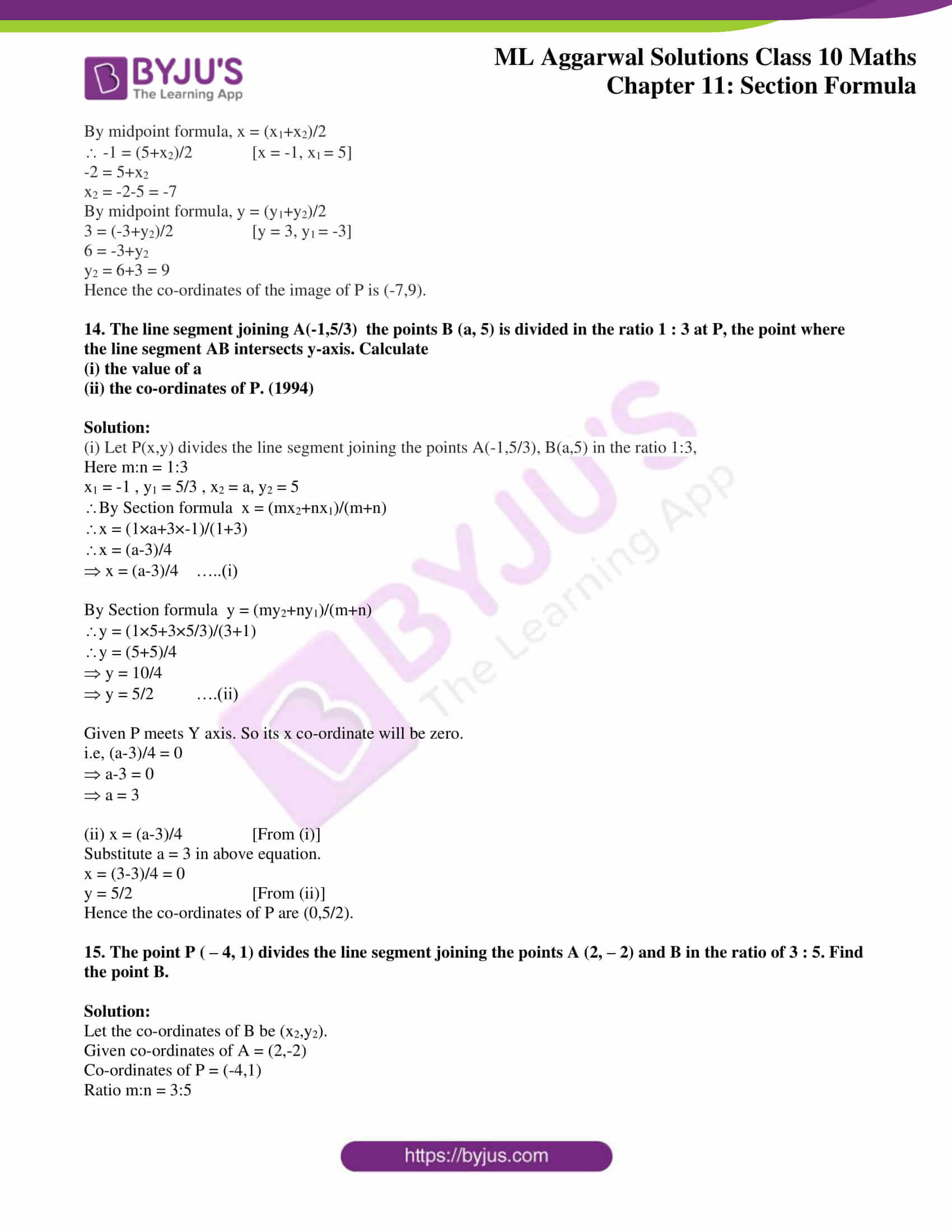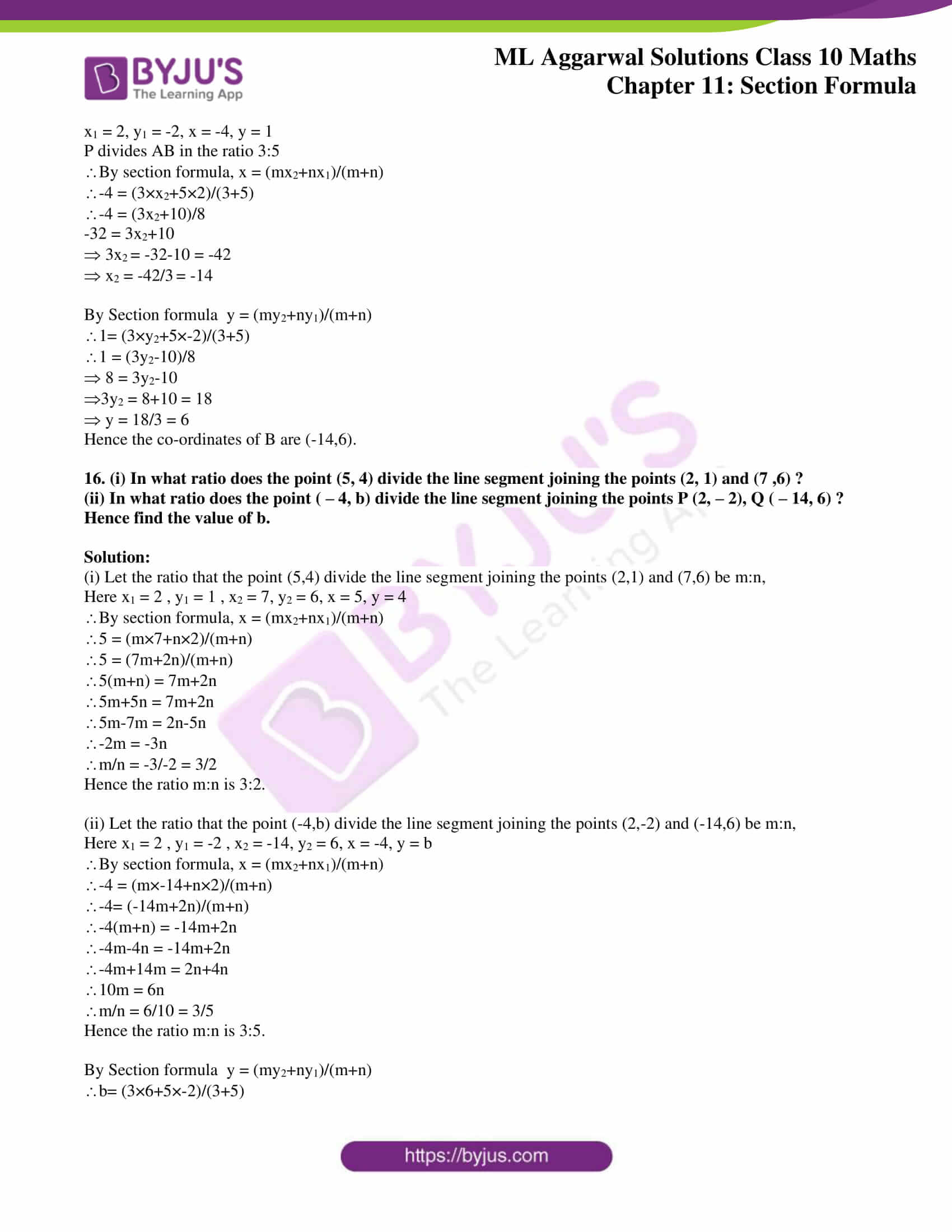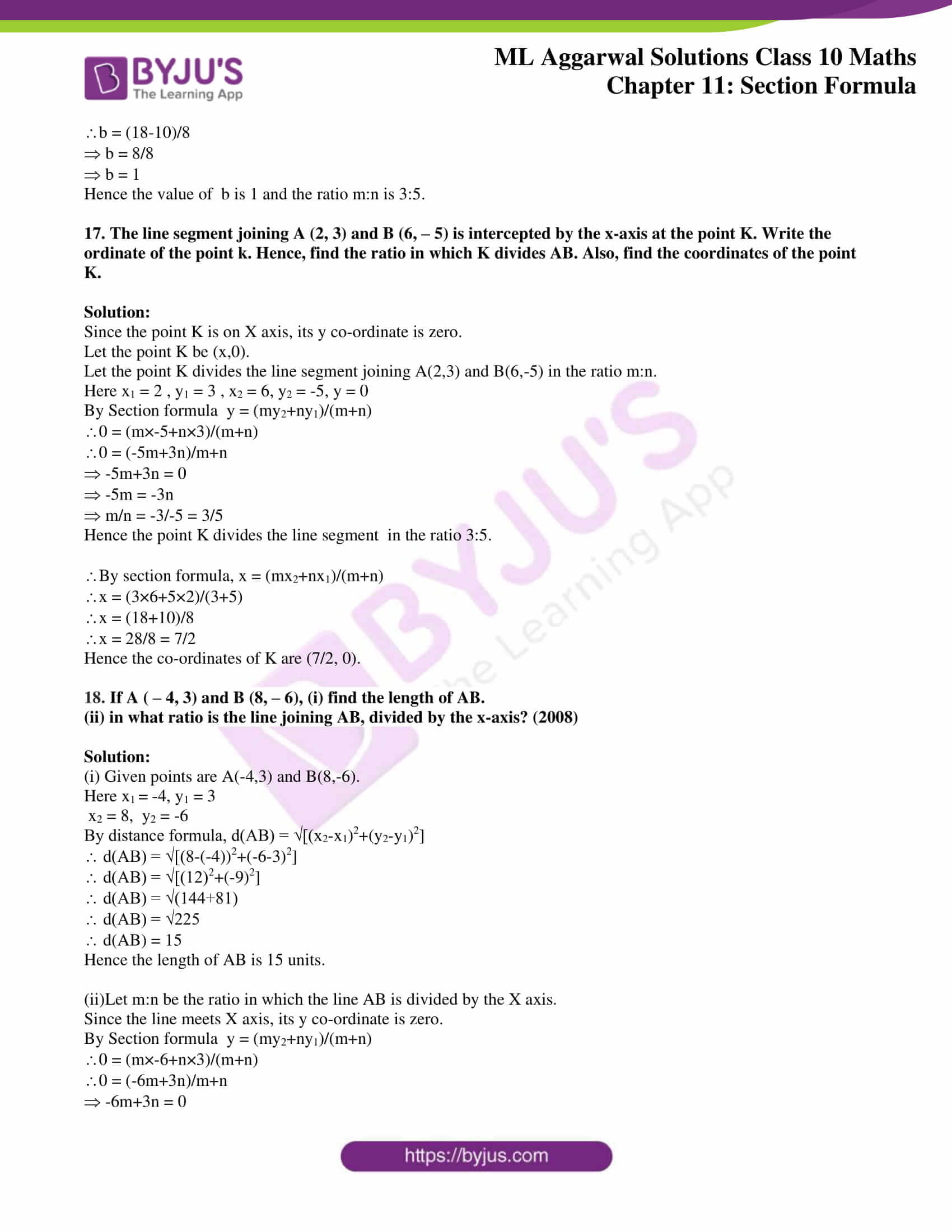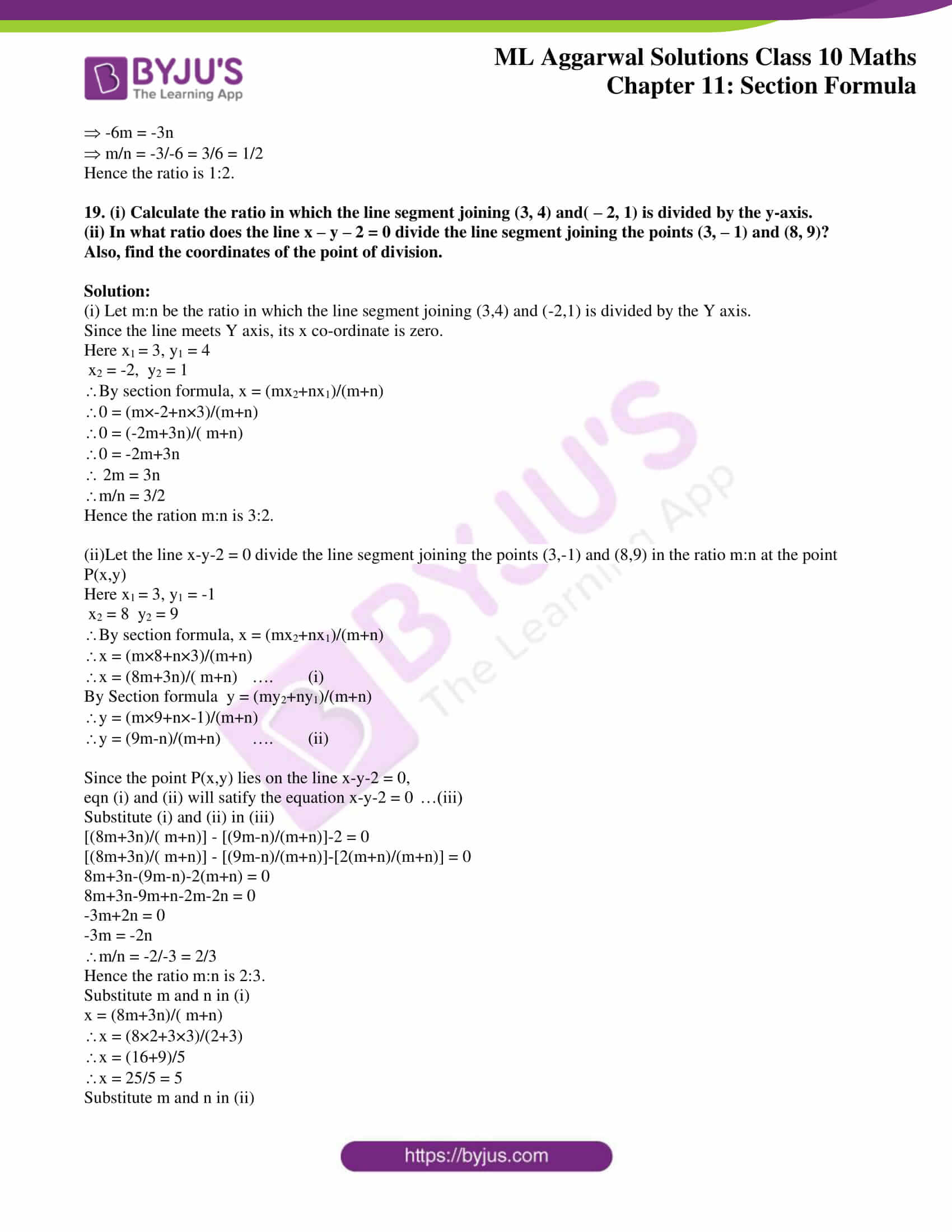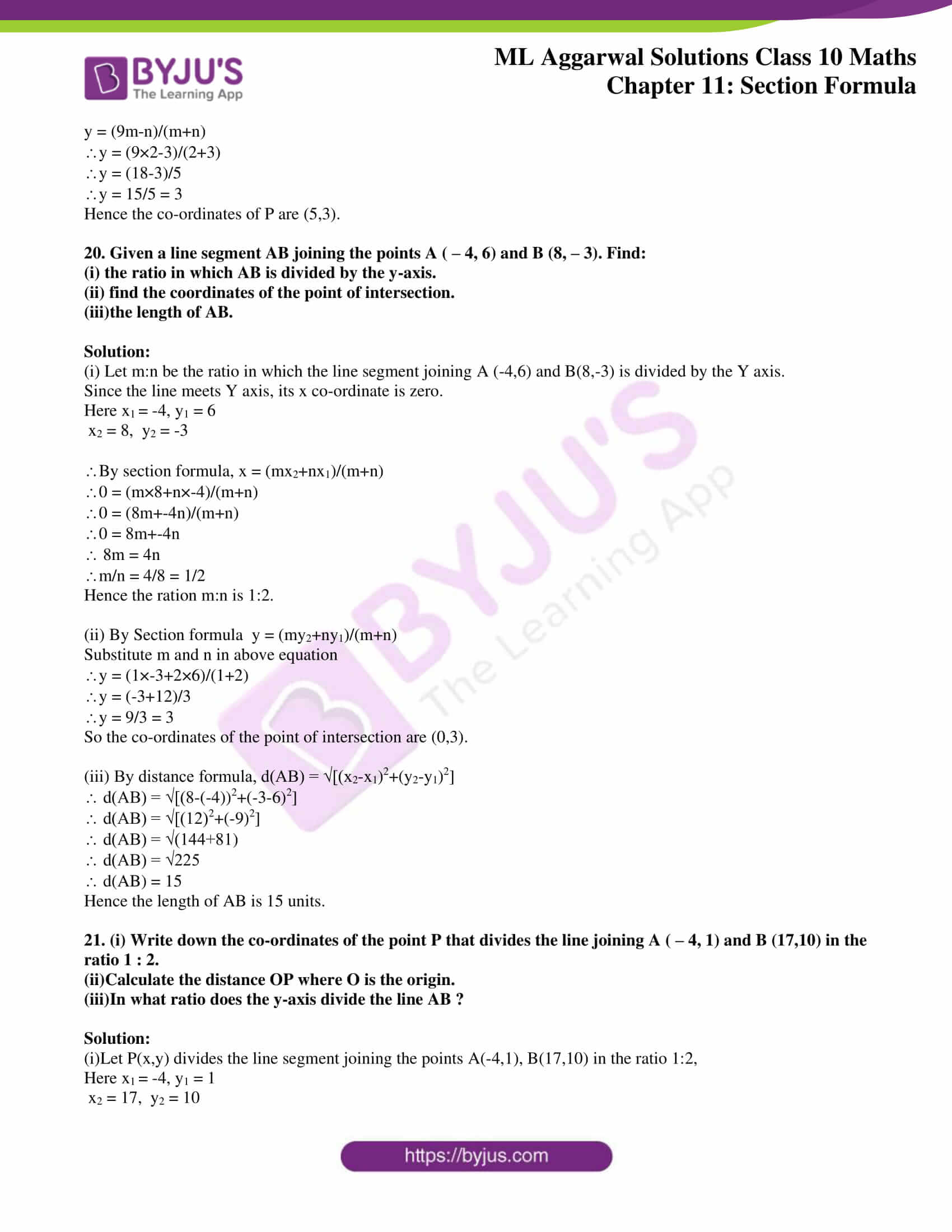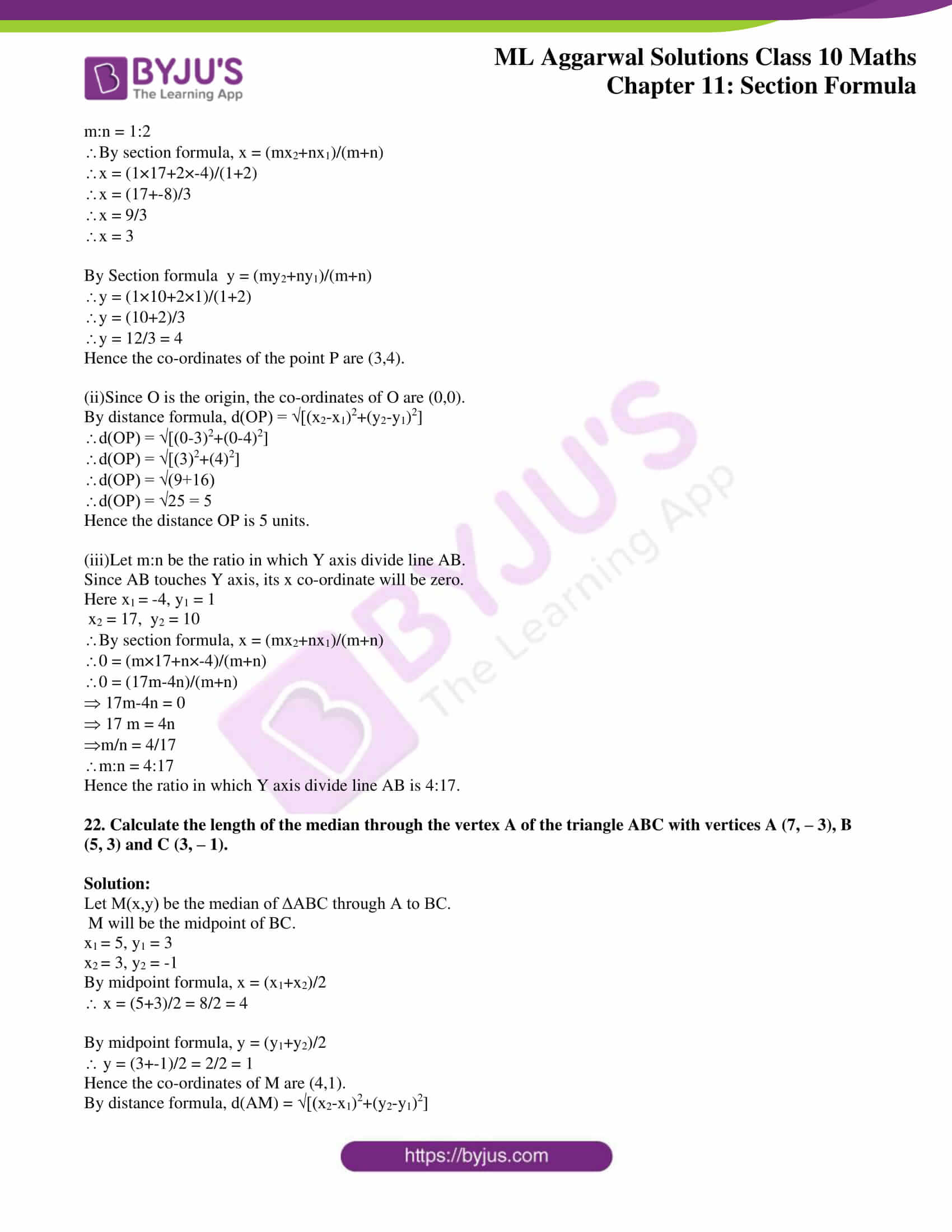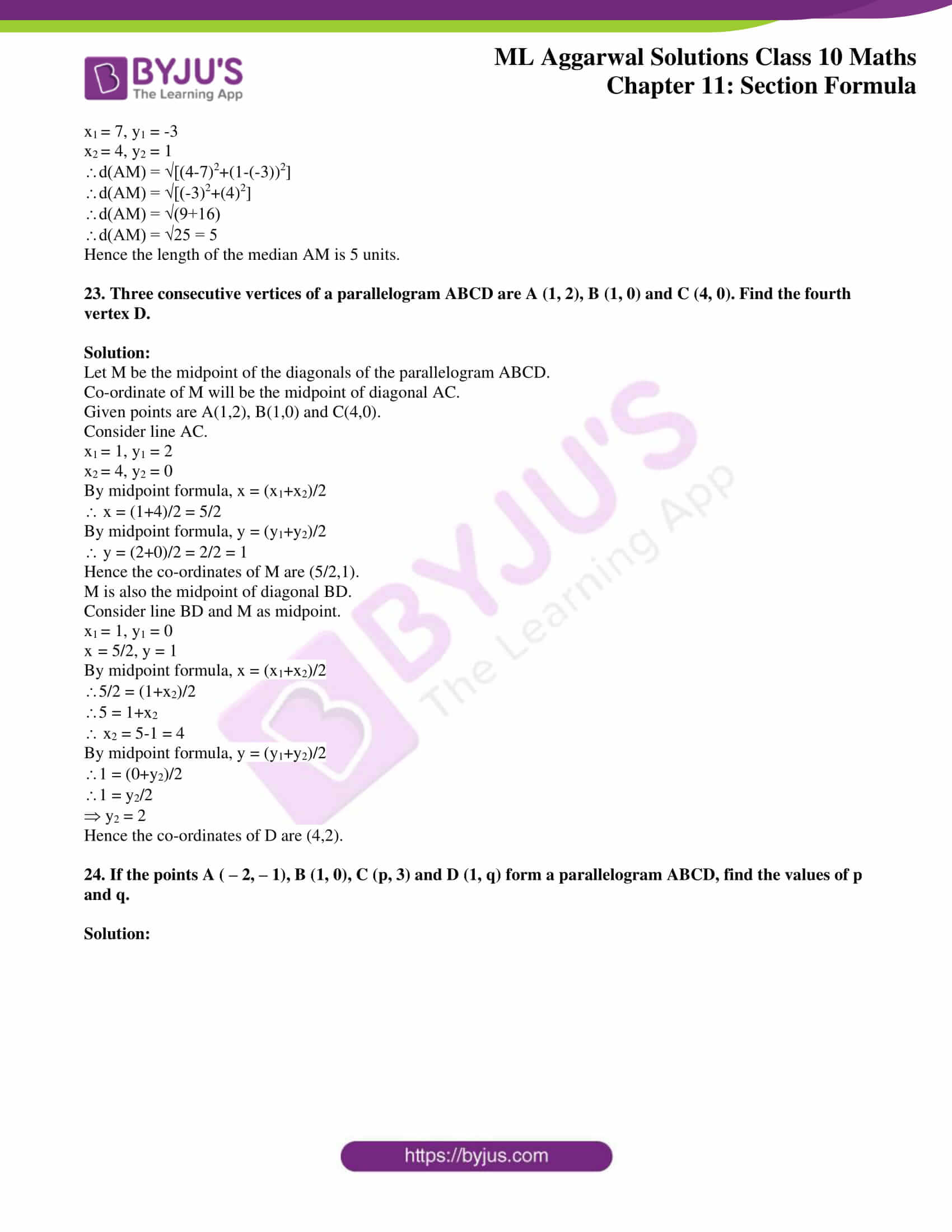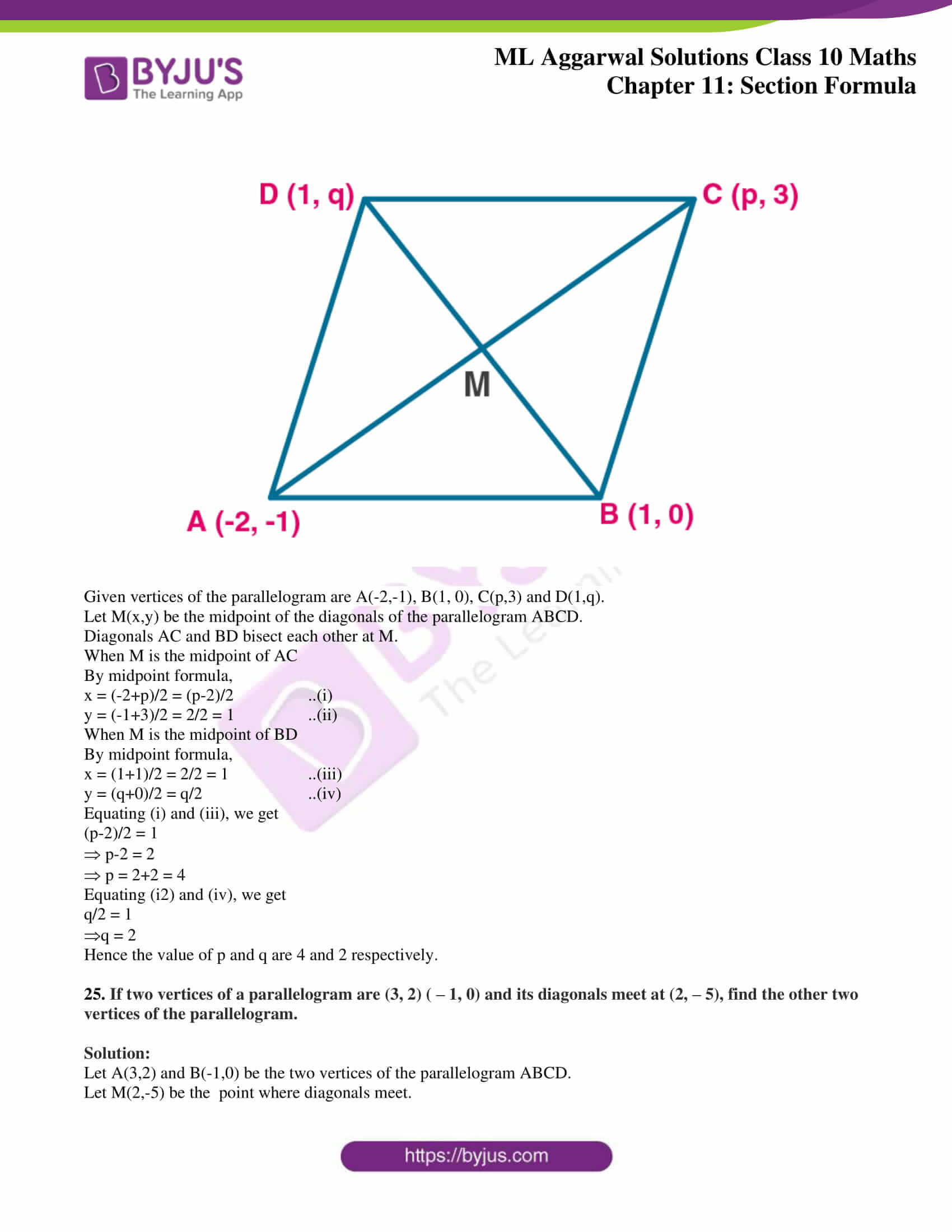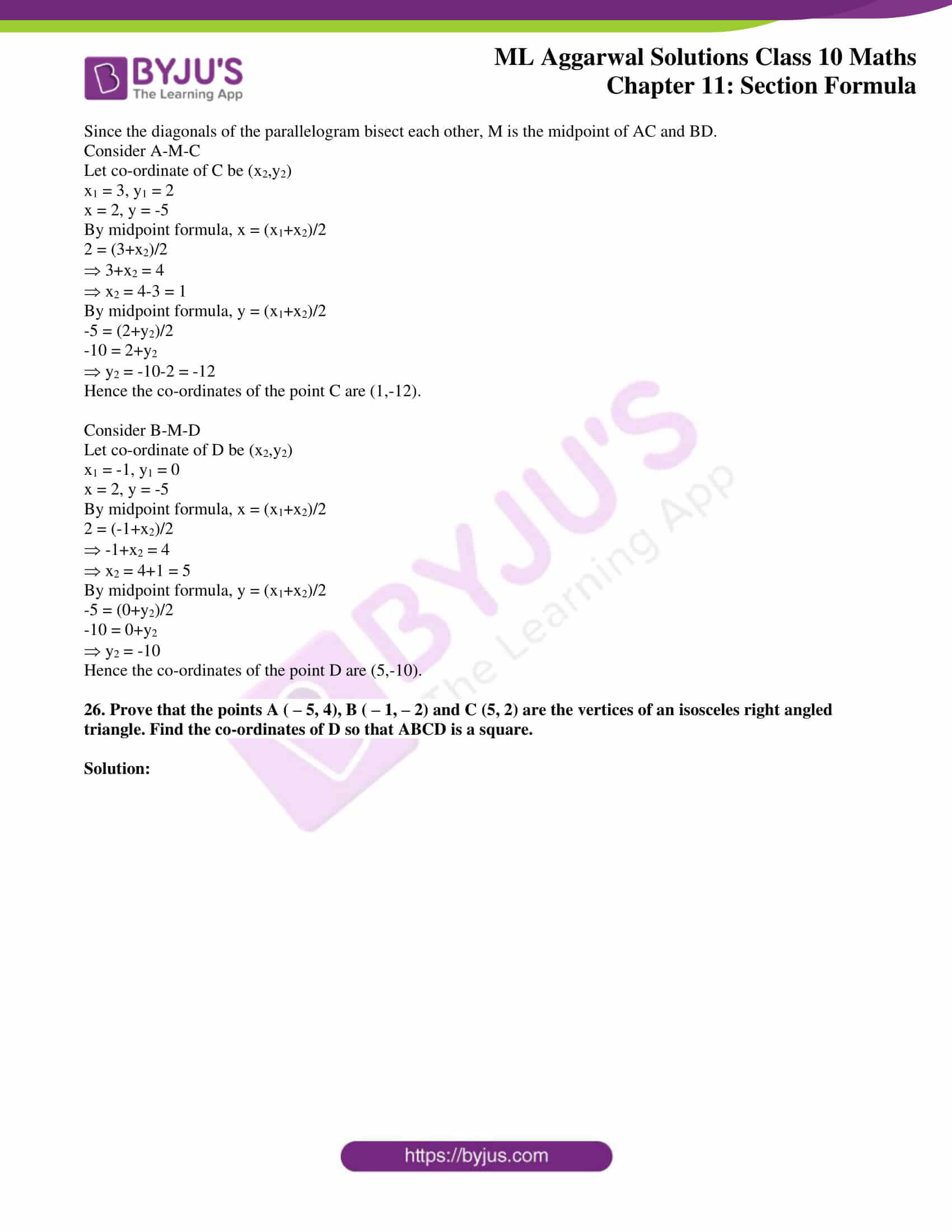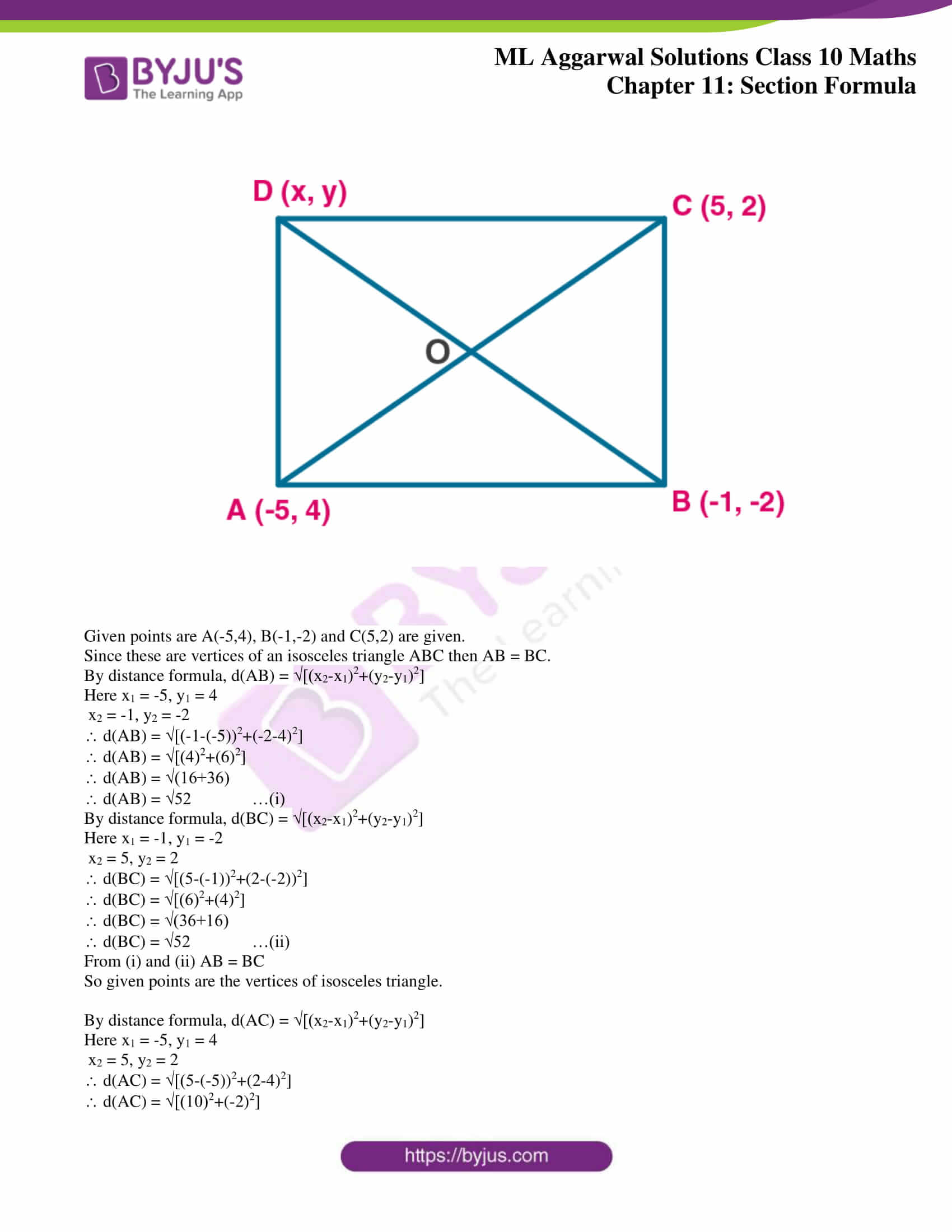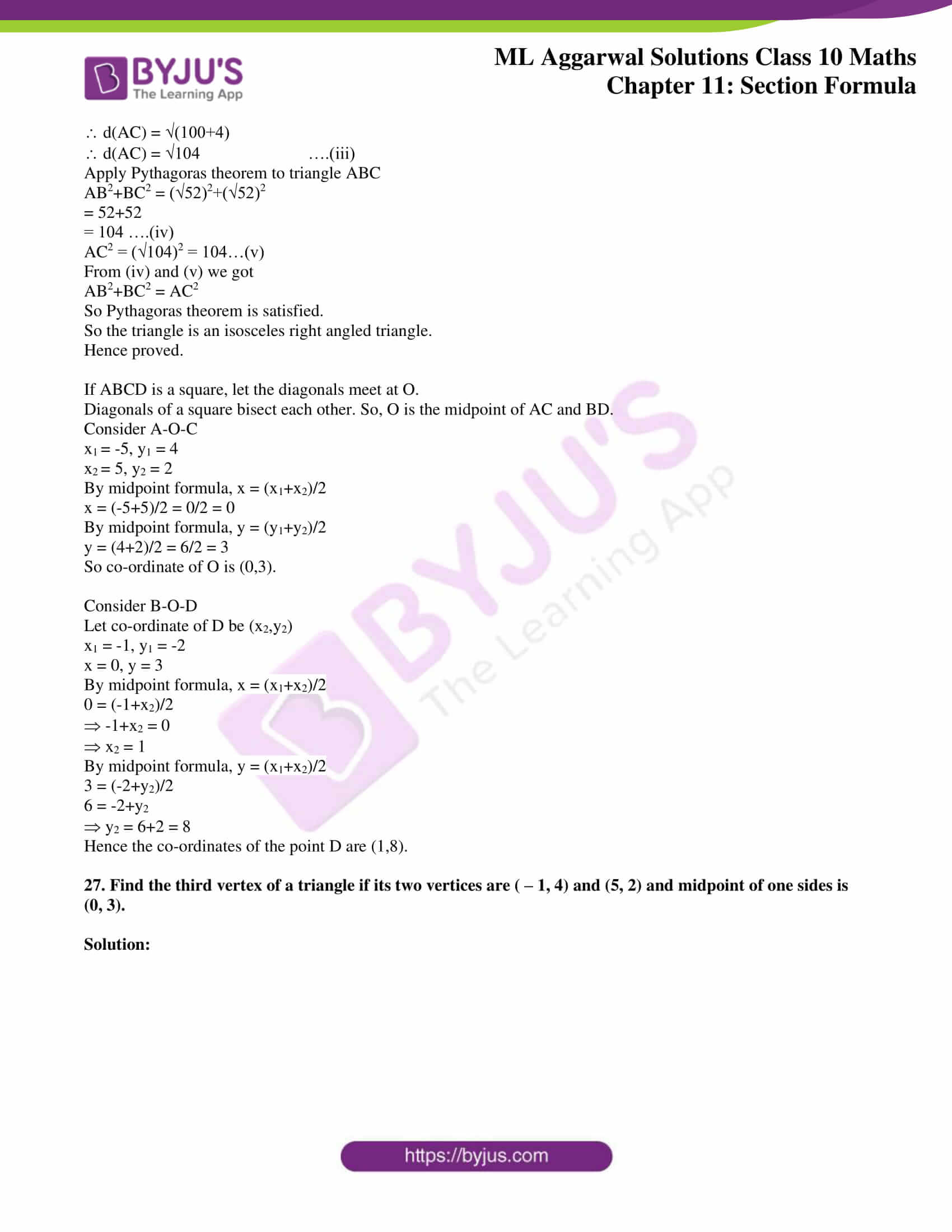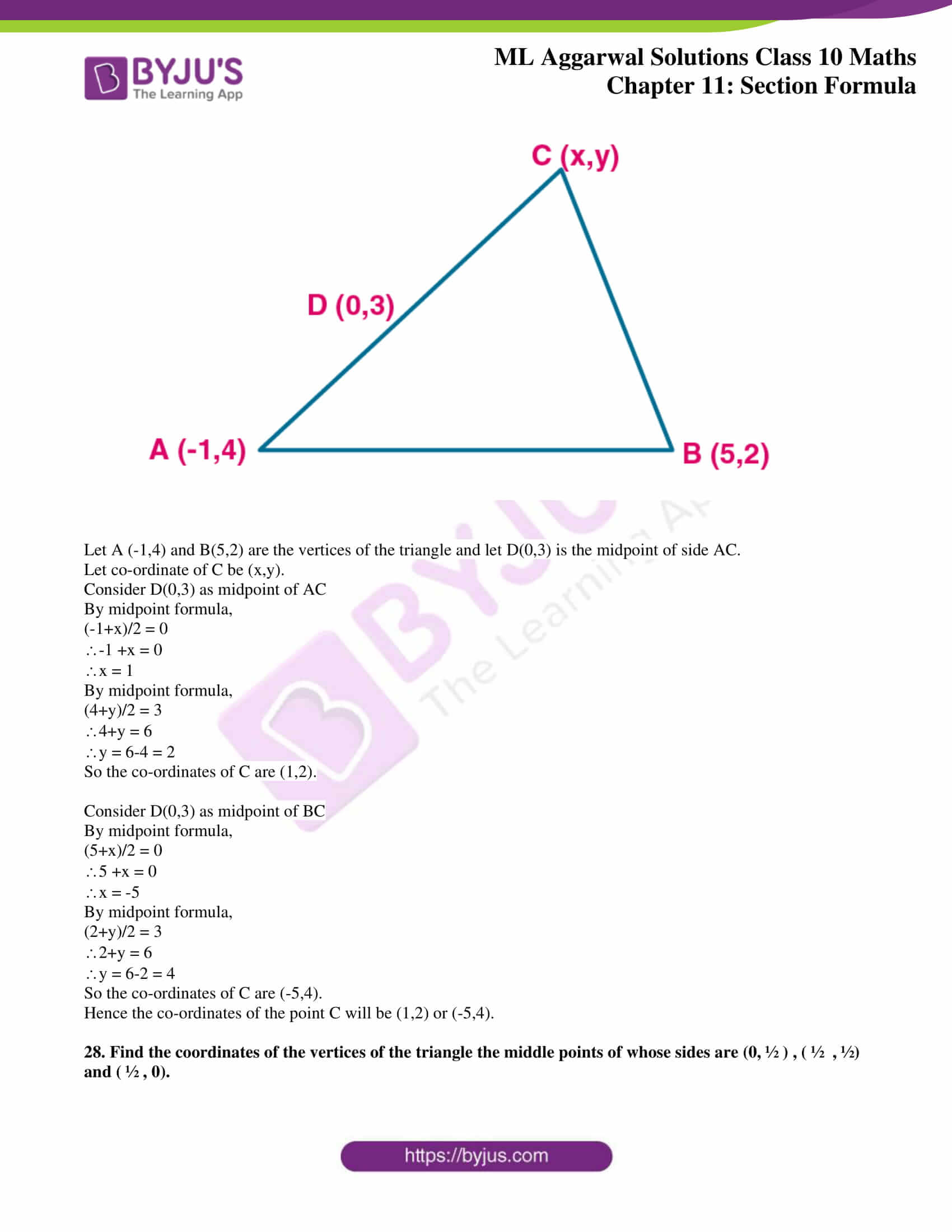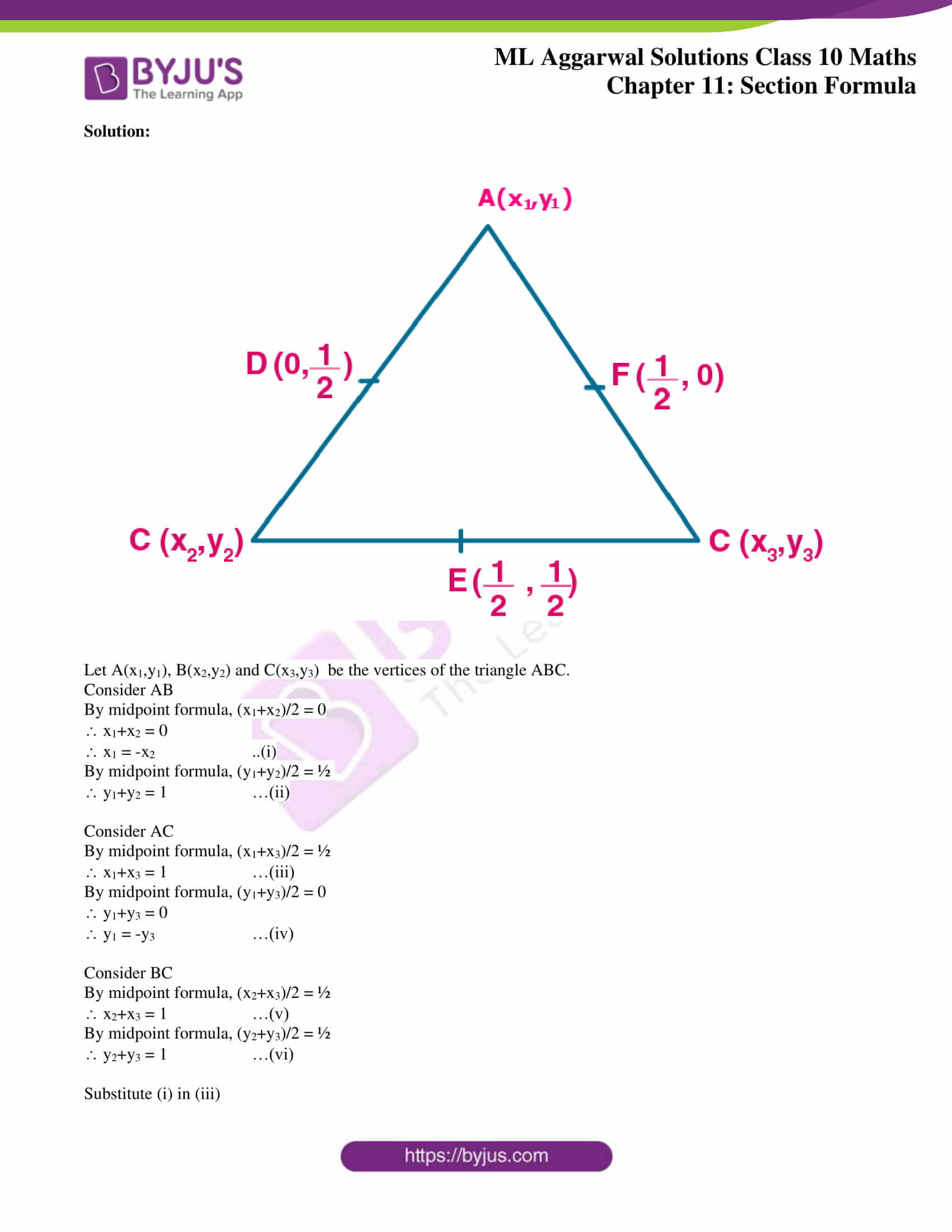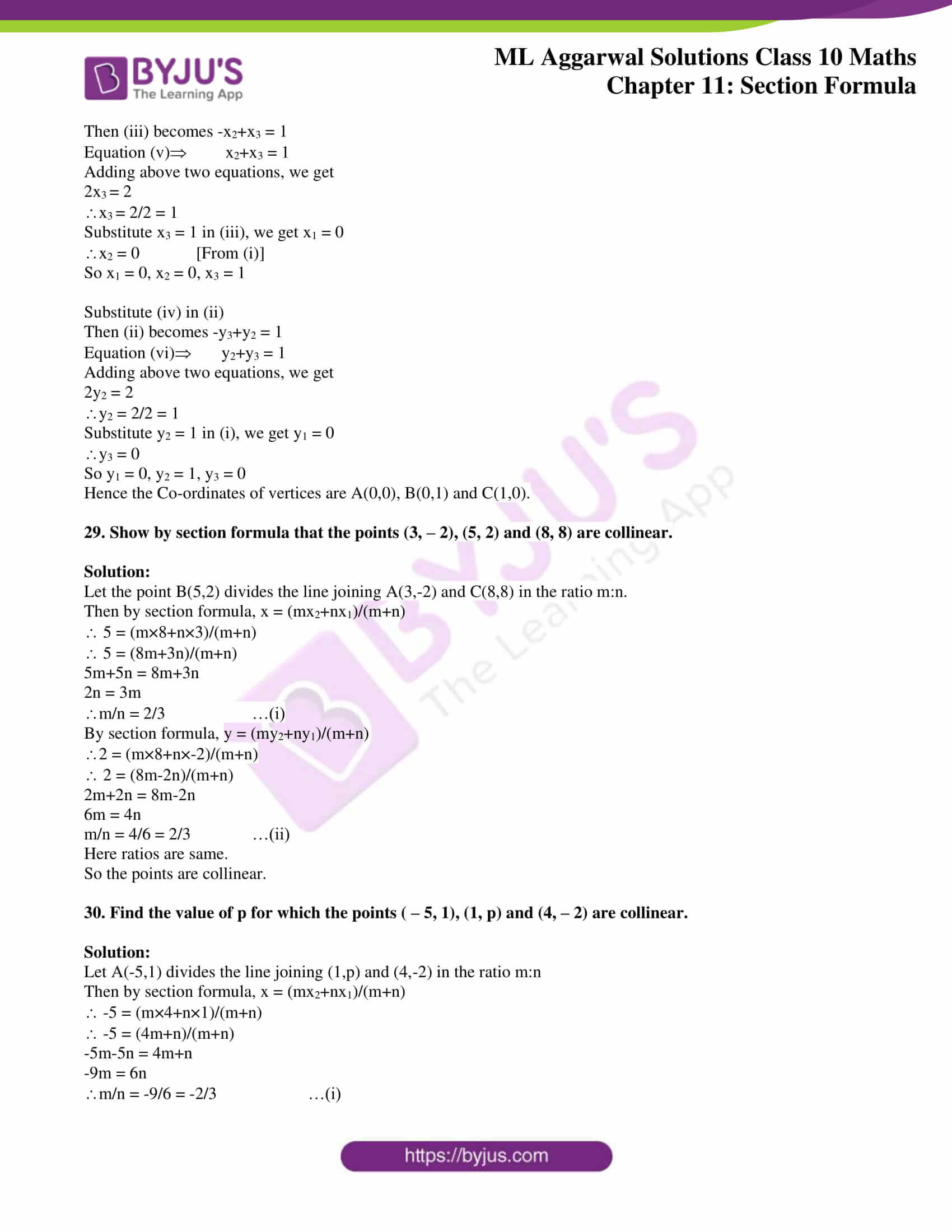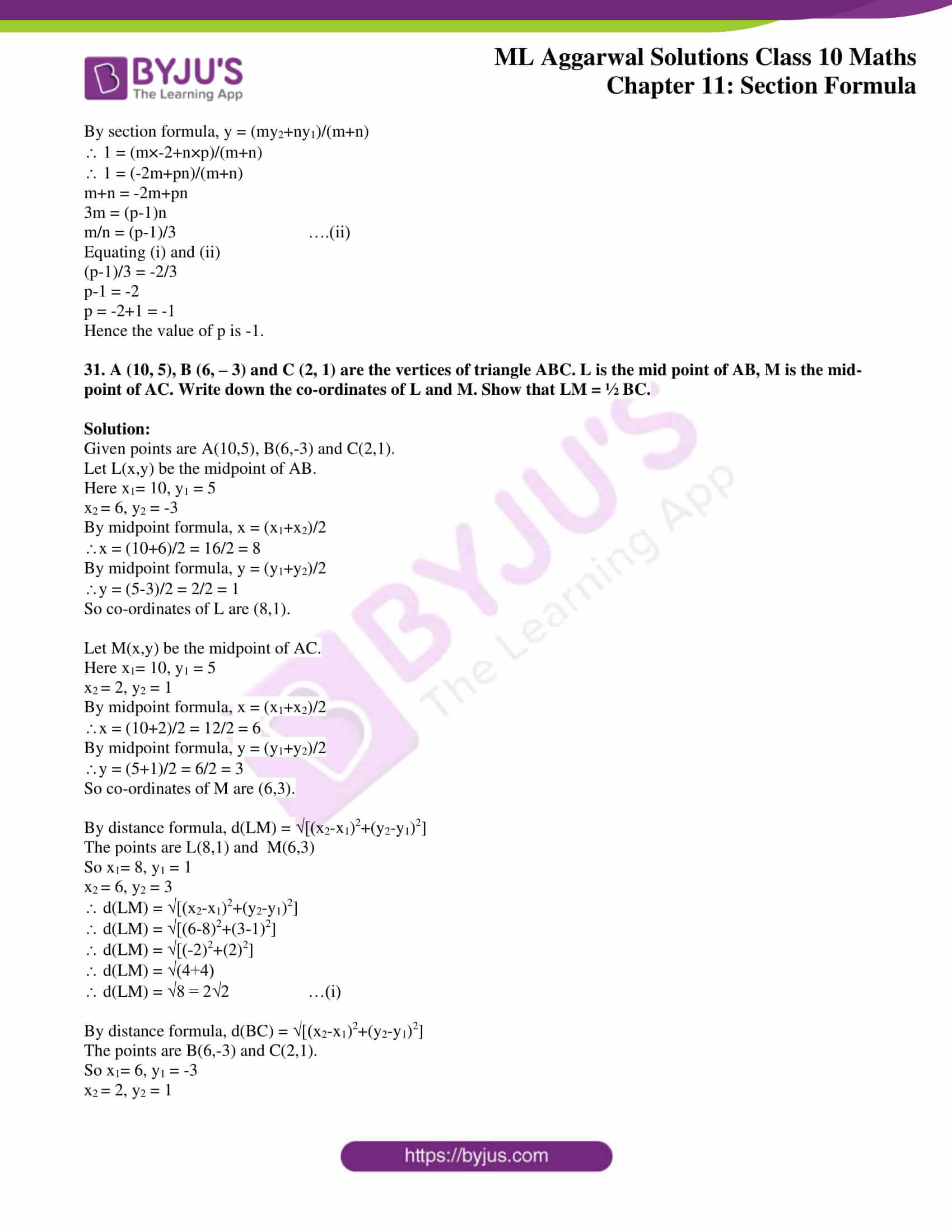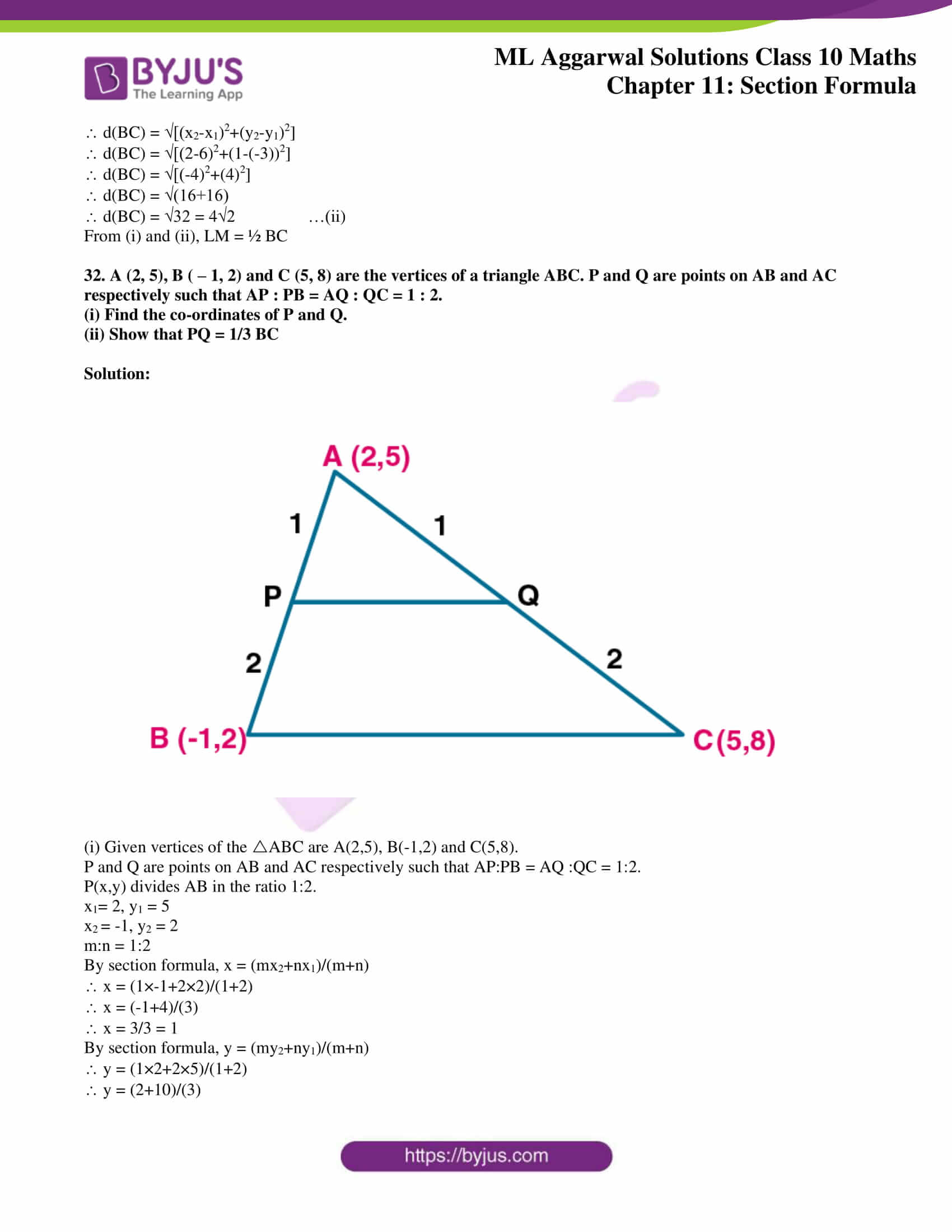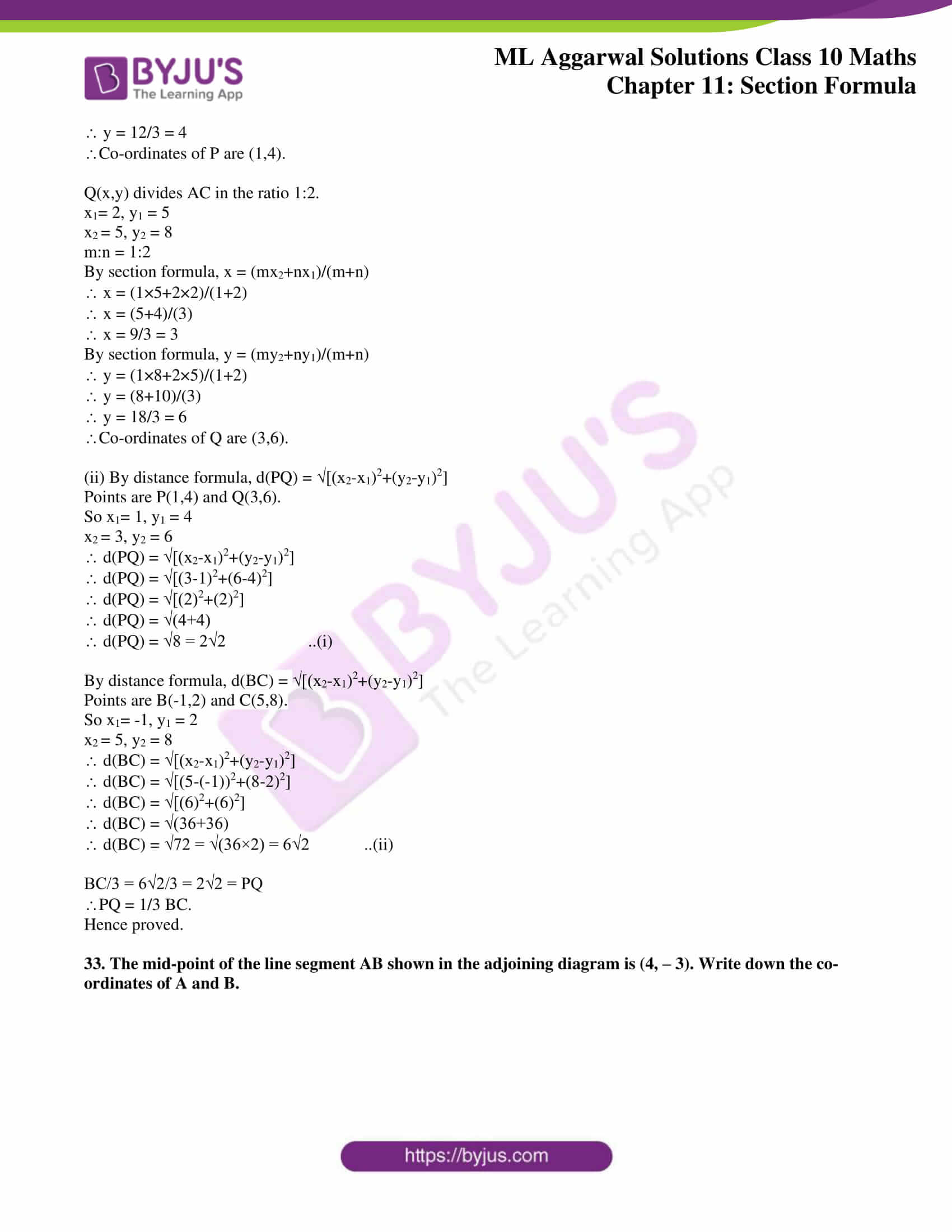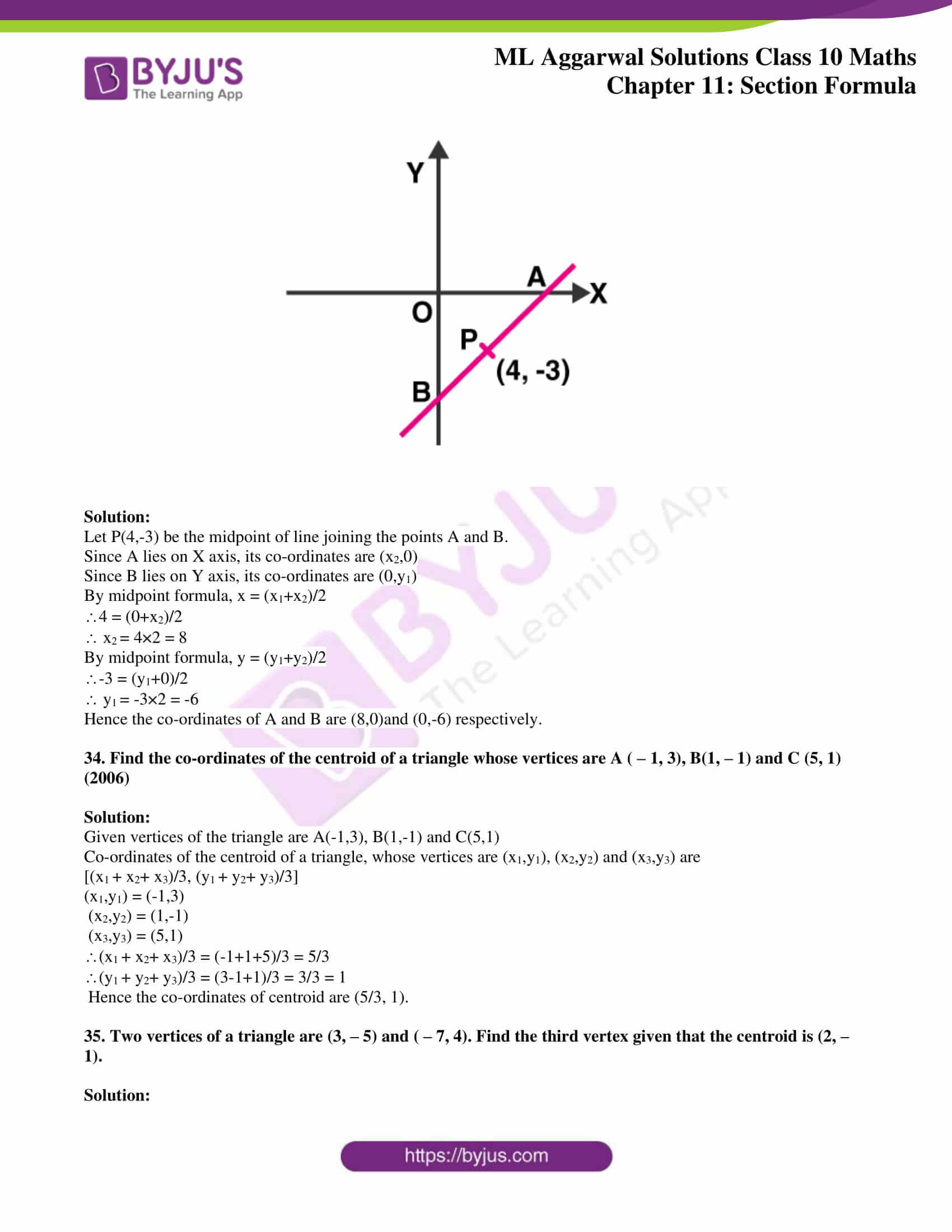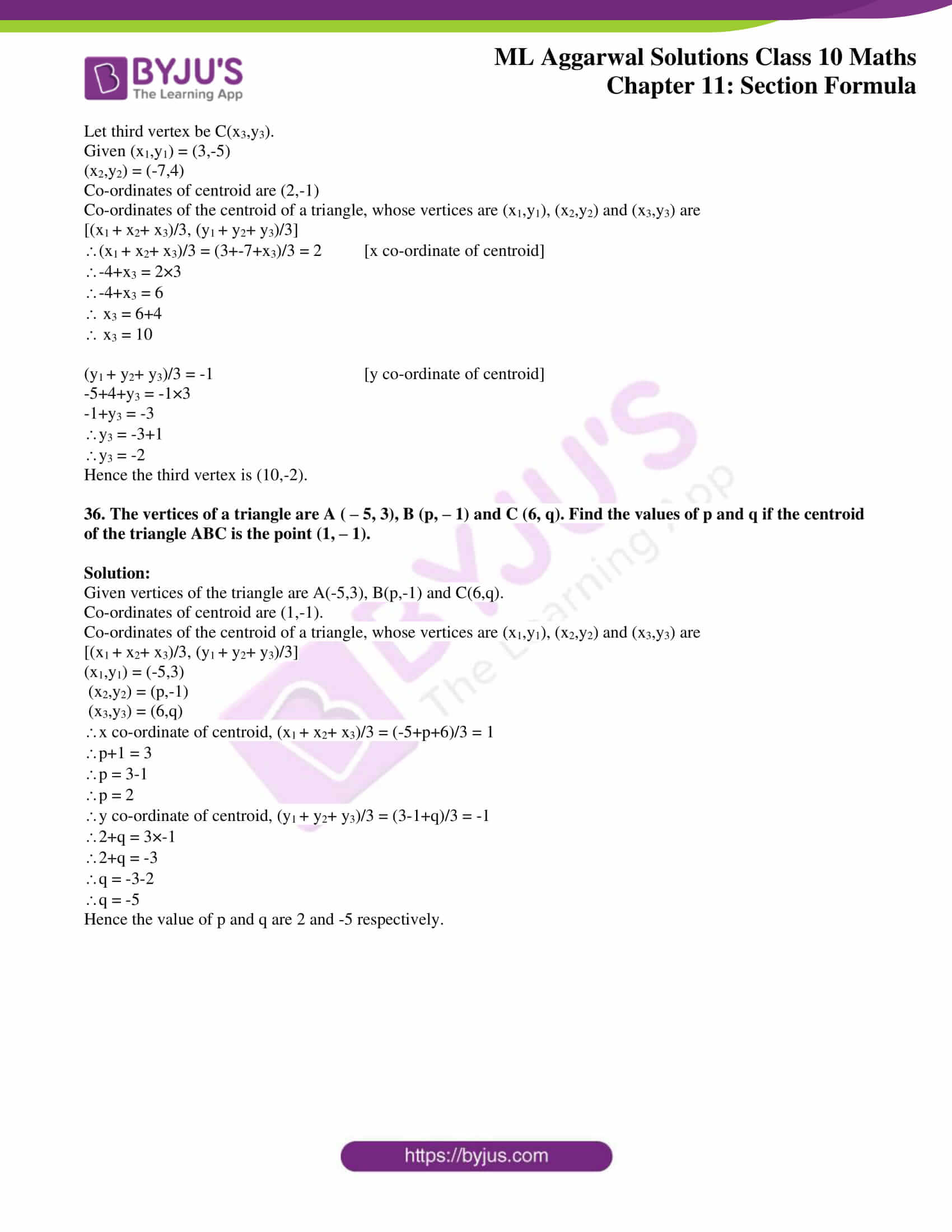### Access answers to ML Aggarwal Solutions for Class 10 Maths Chapter 11 – Section Formula

1. Find the co-ordinates of the mid-point of the line segments joining the following pairs of points:

(i) (2, – 3), ( – 6, 7)

(ii) (5, – 11), (4, 3)

(iii) (a + 3, 5b), (2a – 1, 3b + 4)

Solution:

Co-ordinates of midpoint of line joining the points (x1,y1) and (x2,y2) = {(x1+x2)/2 ,(y1+y2)/2}

(i) Co-ordinates of midpoint of line joining the points (2, -3) and (-6,7) = {(2+-6)/2, (-3+7)/2}

= (-4/2, 4/2)

= (-2, 2)

Hence the co-ordinates of midpoint of line joining the points (2, -3) and (-6,7) is (-2, 2).

(ii) Co-ordinates of midpoint of line joining the points (x1,y1) and (x2,y2) = {(x1+x2)/2 ,(y1+y2)/2}

Co-ordinates of midpoint of line joining the points (5, -11) and (4,3) = {(5+4)/2, (-11+3)/2}

= (9/2, -8/2)

= (9/2, -4)

Hence the co-ordinates of midpoint of line joining the points (5, -11) and (4,3) is (9/2, -4).

(iii) Co-ordinates of midpoint of line joining the points (x1,y1) and (x2,y2) = {(x1+x2)/2 ,(y1+y2)/2}

Co-ordinates of midpoint of line joining the points (a+3, 5b) and (2a-1,3b+4) = {(a+3+2a-1)/2, (5b+3b+4)/2}

= {(3a+2)/2, (8b+4)/2}

= {(3a+2)/2, (4b+2)}

Hence the co-ordinates of midpoint of line joining the points (a+3, 5b) and (2a-1,3b+4) are {(3a+2)/2, (4b+2)}.

2. The co-ordinates of two points A and B are ( – 3, 3) and (12, – 7) respectively. P is a point on the line segment AB such that AP : PB = 2 : 3. Find the co-ordinates of P.

Solution:

Let the co-ordinates of P(x, y) divides AB in the ratio m:n.

A(-3,3) and B(12,-7) are the given points.

Given m:n = 2:3

x1 = -3 , y1 = 3 , x2 = 12 , y2 = -7 , m = 2 and n = 3

By Section formula x = (mx2+nx1)/(m+n)

x = (2×12+3×-3)/(2+3)

x = (24-9)/5

x = 15/5

x = 3

By Section formula y = (my2+ny1)/(m+n)

y = (2×-7+3×3)/5

y = (-14+9)/5

y = -5/5

y = -1

Hence the co-ordinate of point P are (3,-1).

3. P divides the distance between A ( – 2, 1) and B (1, 4) in the ratio of 2 : 1. Calculate the co-ordinates of the point P.

Solution:

Let the co-ordinates of P(x, y) divides AB in the ratio m:n.

A(-2,1) and B(1,4) are the given points.

Given m:n = 2:1

x1 = -2 , y1 = 1 , x2 = 1 , y2 = 4 , m = 2 and n = 1

By Section formula x = (mx2+nx1)/(m+n)

x = (2×1+1×-2)/(2+1)

x = (2-2)/3

x = 0/3

x = 0

By Section formula y = (my2+ny1)/(m+n)

y = (2×4+1×1)/(2+1)

y = (8+1)/3

y = 9/3

y = 3

Hence the co-ordinate of point P are (0,3).

4. (i) Find the co-ordinates of the points of trisection of the line segment joining the point (3, – 3)

and (6, 9).

(ii) The line segment joining the points (3, – 4) and (1, 2) is trisected at the points P and Q. If the coordinates of P and Q are (p, – 2) and (5/3, q) respectively, find the values of p and q.

Solution: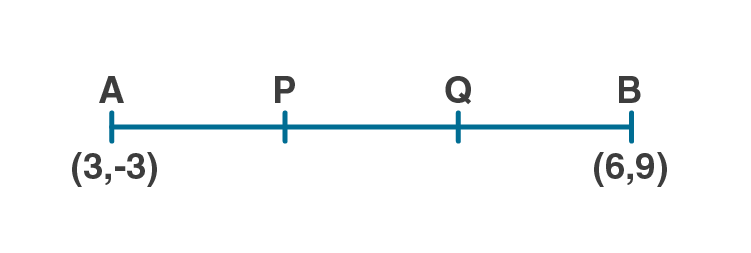Let P and Q be the points of trisection of AB

i.e., AP = PQ = QB

Given A(3,-3) and B(6,9)

x1 = 3, y1 = -3, x2 = 6, y2 = 9

P(x, y) divides AB internally in the ratio 1 : 2.

m:n = 1:2

By applying the section formula, the coordinates of P are as follows.

By Section formula x = (mx2+nx1)/(m+n)

x = (1×6+2×3)/(1+2)

x = (6+6)/3

x = 12/3

x = 4

By Section formula y = (my2+ny1)/(m+n)

y = (1×9+2×-3)/(2+1)

y = (9-6)/3

y = 3/3

y = 1

Hence the co-ordinate of point P are (4,1).

Now, Q also divides AB internally in the ratio 2 : 1.

m:n = 2:1

By applying the section formula, the coordinates of P are as follows.

By Section formula x = (mx2+nx1)/(m+n)

x = (2×6+1×3)/(1+2)

x = (12+3)/3

x = 15/3

x = 5

By Section formula y = (my2+ny1)/(m+n)

y = (2×9+1×-3)/(2+1)

y = (18-3)/3

y = 15/3

y = 5

Hence the co-ordinate of point Q are (5,5).

(ii) Let P(p,-2) and Q(5/3, q) be the points of trisection of AB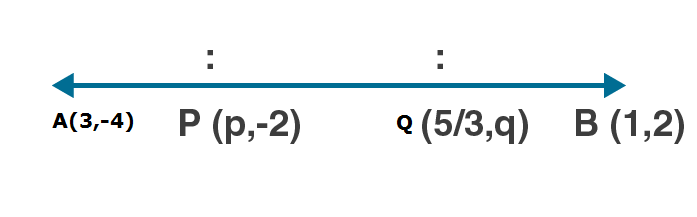i.e., AP = PQ = QB

Given A(3,-4) and B(1,2)

x1 = 3, y1 = -4, x2 = 1, y2 = 2

P(p, -2) divides AB internally in the ratio 1 : 2.

By Section formula x = (mx2+nx1)/(m+n)

p = (1×1+2×3)/(1+2)

p = (1+6)/3

p = 7/3

Now, Q also divides AB internally in the ratio 2 : 1.

m:n = 2:1

Q(5/3, q) divides AB internally in the ratio 2 : 1.

By Section formula y = (my2+ny1)/(m+n)

q = (2×2+1×-4)/(2+1)

q = (4-4)/3

q = 0/3

q = 0

Hence the value of p and q are 7/3 and 0 respectively.

5. (i) The line segment joining the points A (3, 2) and B (5, 1) is divided at the point P in the ratio 1 : 2 and it lies on the line 3x – 18y + k = 0. Find the value of k.

(ii) A point P divides the line segment joining the points A (3, – 5) and B ( – 4, 8) such that AP/PB = k/1  If P lies on the line x + y = 0, then find the value of k.

Solution:

(i) Let the co-ordinates of P(x, y) divides AB in the ratio m:n.

A(3,2) and B(5,1) are the given points.

Given m:n = 1:2

x1 = 3 , y1 = 2 , x2 = 5 , y2 = 1 , m = 1 and n = 2

By Section formula x = (mx2+nx1)/(m+n)

x = (1×5+2×3)/(1+2)

x = (5+6)/3

x = 11/3

By Section formula y = (my2+ny1)/(m+n)

y = (1×1+2×2)/(1+2)

y = (1+4)/3

y = 5/3

Given P lies on the line 3x-18y+k = 0

Substitute x and y in above equation

3×(11/3)-18×(5/3)+k = 0

11-30+k = 0

-19+k = 0

k = 19

Hence the value of k is 19.

(ii) Let the co-ordinates of P(x, y) divides AB in the ratio m:n.

A(3,-5) and B(-4,8) are the given points.

Given AP/PB = k/1

m:n = k:1

x1 = 3 , y1 = -5 , x2 = -4 , y2 = 8 , m = k and n = 1

By Section formula x = (mx2+nx1)/(m+n)

x = (k×-4+1×3)/(k+1)

x = (-4k+3)/(k+1)

x = (-4k+3)/(k+1)

By Section formula y = (my2+ny1)/(m+n)

y = (k×8+1×-5)/(k+1)

y = (-4k+3)/(k+1)

Co-ordinate of P is ((-4k+3)/(k+1), (8k-5)/(k+1))

Given P lies on line x+y = 0

Substitute value of x and y in above equation

(-4k+3)/(k+1) + (8k-5)/(k+1) = 0

(-4k+3) + (8k-5) = 0

4k-2 = 0

4k = 2

k = 2/4 = ½

Hence the value of k is ½ .

6. Find the coordinates of the point which is three-fourth of the way from A (3, 1) to B ( – 2, 5).

Solution: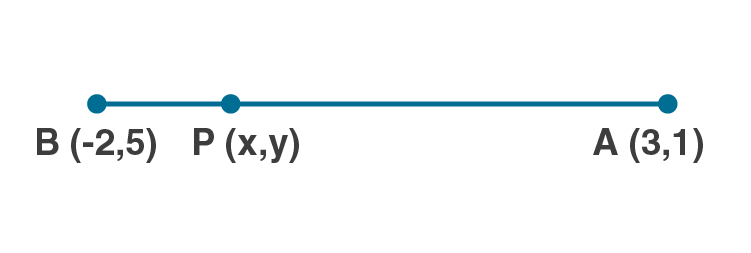Let P be the point which is three-fourth of the way from A(3,1) to B(-2,5).

AP/AB = 3/ 4

AB = AP+PB

AP/AB = AP/(AP+PB) = 3/4

4AP = 3AP+3PB

4AP-3AP = 3PB

AP = 3PB

AP/PB = 3/1

The ratio m:n = 3:1

x1 = 3 , y1 = 1 , x2 = -2, y2 = 5

By Section formula x = (mx2+nx1)/(m+n)

x = (3×-2+1×3)/(3+1)

x = (-6+3)/4

x = -3/4

By Section formula y = (my2+ny1)/(m+n)

y = (3×5+1×1)/(3+1)

y = (15+1)/4

y = 16/4

y = 4

Hence the co-ordinates of P are (-3/4, 4).

7. Point P (3, – 5) is reflected to P’ in the x- axis. Also P on reflection in the y-axis is mapped as P”.

(i) Find the co-ordinates of P’ and P”.

(ii) Compute the distance P’ P”.

(iii) Find the middle point of the line segment P’ P”.

(iv) On which co-ordinate axis does the middle point of the line segment P P” lie ?

Solution:

(i) The image of P(3,-5) when reflected in X-axis will be (3,5).

When you reflect a point across the X-axis, the x-coordinate remains the same,

but the y-coordinate is transformed into its opposite (its sign is changed).

Co-ordinates of P’ = (3,5)

Image of P(3,-5) when reflected in Y axis will be (-3,-5).

When you reflect a point across the Y-axis, the y-coordinate remains the same,

but the x-coordinate is transformed into its opposite (its sign is changed)

Co-ordinates of P’’ = (-3,-5)

(ii)Let P’(x1, y1) and P’’(x2 , y2) be the given points

By distance formula d(P’,P’’) = √[(x2-x1)2+(y2-y1)2]

Co-ordinates of P’ = (3,5)

Co-ordinates of P’’ = (-3,-5)

Here x1 = 3, y1 = 5 , x2 = -3, y2 = -5

d(P’,P’’) = √[(-3-3)2+(-5-5)2]

= √[(-6)2+(-10)2]

= √(36+100)

= √136

= √(4×34)

= 2√34

Hence the distance between P’ and P’’ is 2√34 units.

(iii) Co-ordinates of P’ = (3,5)

Co-ordinates of P’’ = (-3,-5)

Here x1 = 3, y1 = 5 , x2 = -3, y2 = -5

Let Q(x,y) be the midpoint of P’P’’

By midpoint formula,

x = (x1+x2)/2

y = (y1+y2)/2

x = (3+-3)/2 = 0/2 = 0

y = (5+-5)/2 = 0/2 = 0

Hence the co-ordinate of midpoint of P’P’’ is (0,0) .

(iv) Co-ordinates of P = (3,-5)

Co-ordinates of P’’ = (-3,-5)

Here x1 = 3, y1 = -5 , x2 = -3, y2 = -5

Let R(x,y) be the midpoint of PP’’

By midpoint formula,

x = (x1+x2)/2

y = (y1+y2)/2

x = (3+-3)/2 = 0/2 = 0

y = (-5+-5)/2 = -10/2 = -5

So the co-ordinate of midpoint of PP’’ is (0,-5) .

Here x co-ordinate is zero.

Hence the point lies on Y-axis.

8. Use graph paper for this question. Take 1 cm = 1 unit on both axes. Plot the points A(3, 0) and B(0, 4).
(i) Write down the co-ordinates of A1, the reflection of A in the y-axis.

(ii) Write down the co-ordinates of B1, the reflection of B in the x-axis.

(iii) Assign the special name to the quadrilateral ABA1B1.
(iv) If C is the midpoint is AB. Write down the co-ordinates of the point C1, the reflection of C in the origin.
(v) Assign the special name to quadrilateral ABC1B1.

Solution: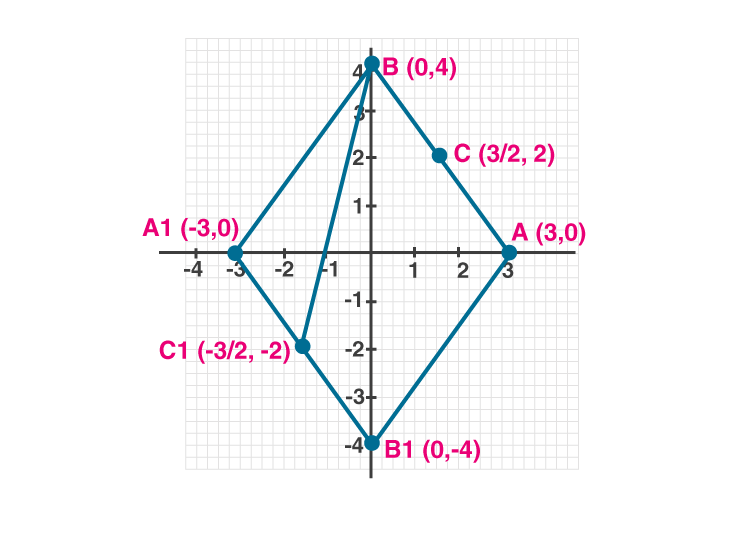(i) Co-ordinates of point A are (3,0).

When you reflect a point across the Y-axis, the y-coordinate remains the same,

but the x-coordinate is transformed into its opposite (its sign is changed)

Hence the reflection of A in the Y axis is (-3,0).

(ii) Co-ordinates of point B are (0,4).

When you reflect a point across the X-axis, the x-coordinate remains the same,

but the y-coordinate is transformed into its opposite (its sign is changed).

Hence the reflection of B in the X-axis is (0,-4)

(iii) The quadrilateral ABA1B1 will be a rhombus.

(iv) Let C be midpoint of AB.

Co-ordinate of C = ((3+0)/2 , (0+4)/2) = (3/2, 2) [Midpoint formula]

In a point reflection in the origin, the image of the point (x,y) is the point (-x,-y).

Hence the reflection of C in the origin is (-3/2, -2)

Hence the quadrilateral ABC1B1 is a trapezium.

9. The line segment joining A ( – 3, 1) and B (5, – 4) is a diameter of a circle whose centre is C. find the co-ordinates of the point C. (1990)

Solution:

Given Co-ordinates of A = (-3,1)

Co-ordinates of B = (5,-4)

Here x1 = -3, y1 = 1 , x2 = 5, y2 = -4

Let C(x,y) be the midpoint of AB

By midpoint formula,

x = (x1+x2)/2

y = (y1+y2)/2

x = (-3+5)/2 = 2/2 = 1

y = (1+-4)/2 = -3/2

Hence the co-ordinate of midpoint of AB is C(1,-3/2) .

10. The mid-point of the line segment joining the points (3m, 6) and ( – 4, 3n) is (1, 2m – 1). Find the values of m and n.

Solution:

Let the midpoint of line joining the points A(3m,6) and B(-4,3n) be C(1,2m-1).

Here x1 = 3m, y1 = 6 , x2 = -4, y2 = 3n

x = 1 , y = 2m-1

By Midpoint formula,

x = (x1+x2)/2

1 = (3m+-4)/2

3m-4 = 2

3m = 2+4

3m = 6

m = 6/3 = 2

By Midpoint formula,

y = (y1+y2)/2

2m-1 = (6+3n)/2

4m-2 = 6+3n

Put m = 2 in above equation

4×2-2 = 6+3n

8-2-6 = 3n

3n = 0

n = 0

Hence the value of m and n are 2 and 0 respectively.

11. The co-ordinates of the mid-point of the line segment PQ are (1, – 2). The co-ordinates of P are ( – 3, 2). Find the co-ordinates of Q.(1992)

Solution:

Let the co-ordinates of Q be (x2, y2).

Given co-ordinates of P = (-3,2)

Co-ordinates of midpoint = (1,-2)

Here x1 = -3, y1 = 2 , x = 1 , y = -2

By Midpoint formula,

x = (x1+x2)/2

1 = (-3+x2)/2

2 = -3+x2

x2 = 2+3 = 5

By Midpoint formula,

y = (y1+y2)/2

-2 = (2+y2)/2

-4 = 2+y2

y2 = -4-2

y2 = -6

Hence the co-ordinates of Q are (5,-6).

12. AB is a diameter of a circle with centre C ( – 2, 5). If point A is (3, – 7). Find:

(i) the length of radius AC.

(ii) the coordinates of B.

Solution: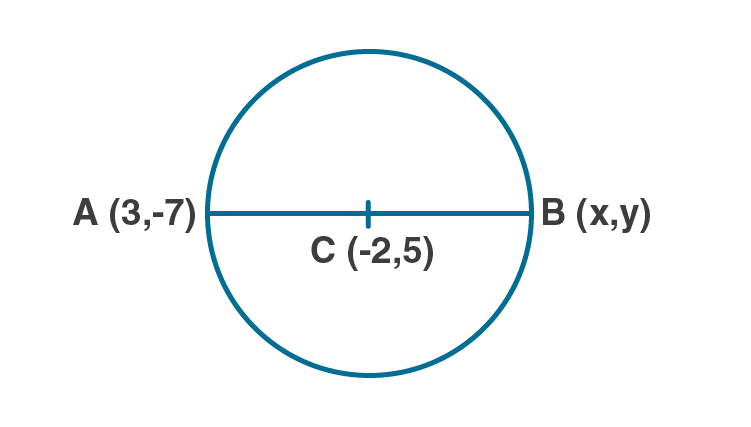(i) Length of radius AC = d(A,C)

Co-ordinates of A = (3,-7)

Co-ordinates of C = (-2,5)

Here x1 = 3, y1 = -7, x2 = -2, y2 = 5

By distance formula, d(A,C) = √[(x2-x1)2+(y2-y1)2]

= √[(-2-3)2+(5-(-7))2]

= √[(-5)2+(12)2]

= √[25+144]

= √169

= 13

Hence the radius is 13 units.

(ii)Given AB is the diameter and C is the centre of the circle.

By midpoint formula, -2 = (x+3)/2

-4 = x+3

x = -4-3 = -7

By midpoint formula, 5 = (-7+y)/2

10 = -7+y

y = 10+7 = 17

Hence the co-ordinates of B are (-7,17).

13. Find the reflection (image) of the point (5, – 3) in the point ( – 1, 3).

Solution:

Let the co-ordinates of the image of the point P(5,-3) be

P1(x, y) in the point (-1, 3) then the point (-1, 3) will be the midpoint of PP1.

By midpoint formula, x = (x1+x2)/2

-1 = (5+x2)/2 [x = -1, x1 = 5]

-2 = 5+x2

x2 = -2-5 = -7

By midpoint formula, y = (y1+y2)/2

3 = (-3+y2)/2 [y = 3, y1 = -3]

6 = -3+y2

y2 = 6+3 = 9

Hence the co-ordinates of the image of P is (-7,9).

14. The line segment joining A(-1,5/3)  the points B (a, 5) is divided in the ratio 1 : 3 at P, the point where the line segment AB intersects y-axis. Calculate
(i) the value of a
(ii) the co-ordinates of P. (1994)

Solution:

(i) Let P(x,y) divides the line segment joining the points A(-1,5/3), B(a,5) in the ratio 1:3,

Here m:n = 1:3

x1 = -1 , y1 = 5/3 , x2 = a, y2 = 5

By Section formula x = (mx2+nx1)/(m+n)

x = (1×a+3×-1)/(1+3)

x = (a-3)/4

x = (a-3)/4 …..(i)

By Section formula y = (my2+ny1)/(m+n)

y = (1×5+3×5/3)/(3+1)

y = (5+5)/4

y = 10/4

y = 5/2 ….(ii)

Given P meets Y axis. So its x co-ordinate will be zero.

i.e, (a-3)/4 = 0

a-3 = 0

a = 3

(ii) x = (a-3)/4 [From (i)]

Substitute a = 3 in above equation.

x = (3-3)/4 = 0

y = 5/2 [From (ii)]

Hence the co-ordinates of P are (0,5/2).

15. The point P ( – 4, 1) divides the line segment joining the points A (2, – 2) and B in the ratio of 3 : 5. Find the point B.

Solution:

Let the co-ordinates of B be (x2,y2).

Given co-ordinates of A = (2,-2)

Co-ordinates of P = (-4,1)

Ratio m:n = 3:5

x1 = 2, y1 = -2, x = -4, y = 1

P divides AB in the ratio 3:5

By section formula, x = (mx2+nx1)/(m+n)

-4 = (3×x2+5×2)/(3+5)

-4 = (3x2+10)/8

-32 = 3x2+10

3x2 = -32-10 = -42

x2 = -42/3 = -14

By Section formula y = (my2+ny1)/(m+n)

1= (3×y2+5×-2)/(3+5)

1 = (3y2-10)/8

8 = 3y2-10

3y2 = 8+10 = 18

y = 18/3 = 6

Hence the co-ordinates of B are (-14,6).

16. (i) In what ratio does the point (5, 4) divide the line segment joining the points (2, 1) and (7 ,6) ?
(ii) In what ratio does the point ( – 4, b) divide the line segment joining the points P (2, – 2), Q ( – 14, 6) ? Hence find the value of b.

Solution:

(i) Let the ratio that the point (5,4) divide the line segment joining the points (2,1) and (7,6) be m:n,

Here x1 = 2 , y1 = 1 , x2 = 7, y2 = 6, x = 5, y = 4

By section formula, x = (mx2+nx1)/(m+n)

5 = (m×7+n×2)/(m+n)

5 = (7m+2n)/(m+n)

5(m+n) = 7m+2n

5m+5n = 7m+2n

5m-7m = 2n-5n

-2m = -3n

m/n = -3/-2 = 3/2

Hence the ratio m:n is 3:2.

(ii) Let the ratio that the point (-4,b) divide the line segment joining the points (2,-2) and (-14,6) be m:n,

Here x1 = 2 , y1 = -2 , x2 = -14, y2 = 6, x = -4, y = b

By section formula, x = (mx2+nx1)/(m+n)

-4 = (m×-14+n×2)/(m+n)

-4= (-14m+2n)/(m+n)

-4(m+n) = -14m+2n

-4m-4n = -14m+2n

-4m+14m = 2n+4n

10m = 6n

m/n = 6/10 = 3/5

Hence the ratio m:n is 3:5.

By Section formula y = (my2+ny1)/(m+n)

b= (3×6+5×-2)/(3+5)

b = (18-10)/8

b = 8/8

b = 1

Hence the value of b is 1 and the ratio m:n is 3:5.

17. The line segment joining A (2, 3) and B (6, – 5) is intercepted by the x-axis at the point K. Write the ordinate of the point k. Hence, find the ratio in which K divides AB. Also, find the coordinates of the point K.

Solution:

Since the point K is on X axis, its y co-ordinate is zero.

Let the point K be (x,0).

Let the point K divides the line segment joining A(2,3) and B(6,-5) in the ratio m:n.

Here x1 = 2 , y1 = 3 , x2 = 6, y2 = -5, y = 0

By Section formula y = (my2+ny1)/(m+n)

0 = (m×-5+n×3)/(m+n)

0 = (-5m+3n)/m+n

-5m+3n = 0

-5m = -3n

m/n = -3/-5 = 3/5

Hence the point K divides the line segment in the ratio 3:5.

By section formula, x = (mx2+nx1)/(m+n)

x = (3×6+5×2)/(3+5)

x = (18+10)/8

x = 28/8 = 7/2

Hence the co-ordinates of K are (7/2, 0).

18. If A ( – 4, 3) and B (8, – 6), (i) find the length of AB.

(ii) in what ratio is the line joining AB, divided by the x-axis? (2008)

Solution:

(i) Given points are A(-4,3) and B(8,-6).

Here x1 = -4, y1 = 3

x2 = 8, y2 = -6

By distance formula, d(AB) = √[(x2-x1)2+(y2-y1)2]

d(AB) = √[(8-(-4))2+(-6-3)2]

d(AB) = √[(12)2+(-9)2]

d(AB) = √(144+81)

d(AB) = √225

d(AB) = 15

Hence the length of AB is 15 units.

(ii)Let m:n be the ratio in which the line AB is divided by the X axis.

Since the line meets X axis, its y co-ordinate is zero.

By Section formula y = (my2+ny1)/(m+n)

0 = (m×-6+n×3)/(m+n)

0 = (-6m+3n)/m+n

-6m+3n = 0

-6m = -3n

m/n = -3/-6 = 3/6 = 1/2

Hence the ratio is 1:2.

19. (i) Calculate the ratio in which the line segment joining (3, 4) and( – 2, 1) is divided by the y-axis.

(ii) In what ratio does the line x – y – 2 = 0 divide the line segment joining the points (3, – 1) and (8, 9)? Also, find the coordinates of the point of division.

Solution:

(i) Let m:n be the ratio in which the line segment joining (3,4) and (-2,1) is divided by the Y axis.

Since the line meets Y axis, its x co-ordinate is zero.

Here x1 = 3, y1 = 4

x2 = -2, y2 = 1

By section formula, x = (mx2+nx1)/(m+n)

0 = (m×-2+n×3)/(m+n)

0 = (-2m+3n)/( m+n)

0 = -2m+3n

2m = 3n

m/n = 3/2

Hence the ration m:n is 3:2.

(ii)Let the line x-y-2 = 0 divide the line segment joining the points (3,-1) and (8,9) in the ratio m:n at the point P(x,y)

Here x1 = 3, y1 = -1

x2 = 8 y2 = 9

By section formula, x = (mx2+nx1)/(m+n)

x = (m×8+n×3)/(m+n)

x = (8m+3n)/( m+n) …. (i)

By Section formula y = (my2+ny1)/(m+n)

y = (m×9+n×-1)/(m+n)

y = (9m-n)/(m+n) …. (ii)

Since the point P(x,y) lies on the line x-y-2 = 0,

eqn (i) and (ii) will satify the equation x-y-2 = 0 …(iii)

Substitute (i) and (ii) in (iii)

[(8m+3n)/( m+n)] – [(9m-n)/(m+n)]-2 = 0

[(8m+3n)/( m+n)] – [(9m-n)/(m+n)]-[2(m+n)/(m+n)] = 0

8m+3n-(9m-n)-2(m+n) = 0

8m+3n-9m+n-2m-2n = 0

-3m+2n = 0

-3m = -2n

m/n = -2/-3 = 2/3

Hence the ratio m:n is 2:3.

Substitute m and n in (i)

x = (8m+3n)/( m+n)

x = (8×2+3×3)/(2+3)

x = (16+9)/5

x = 25/5 = 5

Substitute m and n in (ii)

y = (9m-n)/(m+n)

y = (9×2-3)/(2+3)

y = (18-3)/5

y = 15/5 = 3

Hence the co-ordinates of P are (5,3).

20. Given a line segment AB joining the points A ( – 4, 6) and B (8, – 3). Find:

(i) the ratio in which AB is divided by the y-axis.

(ii) find the coordinates of the point of intersection.

(iii)the length of AB.

Solution:

(i) Let m:n be the ratio in which the line segment joining A (-4,6) and B(8,-3) is divided by the Y axis.

Since the line meets Y axis, its x co-ordinate is zero.

Here x1 = -4, y1 = 6

x2 = 8, y2 = -3

By section formula, x = (mx2+nx1)/(m+n)

0 = (m×8+n×-4)/(m+n)

0 = (8m+-4n)/(m+n)

0 = 8m+-4n

8m = 4n

m/n = 4/8 = 1/2

Hence the ration m:n is 1:2.

(ii) By Section formula y = (my2+ny1)/(m+n)

Substitute m and n in above equation

y = (1×-3+2×6)/(1+2)

y = (-3+12)/3

y = 9/3 = 3

So the co-ordinates of the point of intersection are (0,3).

(iii) By distance formula, d(AB) = √[(x2-x1)2+(y2-y1)2]

d(AB) = √[(8-(-4))2+(-3-6)2]

d(AB) = √[(12)2+(-9)2]

d(AB) = √(144+81)

d(AB) = √225

d(AB) = 15

Hence the length of AB is 15 units.

21. (i) Write down the co-ordinates of the point P that divides the line joining A ( – 4, 1) and B (17,10) in the ratio 1 : 2.

(ii)Calculate the distance OP where O is the origin.

(iii)In what ratio does the y-axis divide the line AB ?

Solution:

(i)Let P(x,y) divides the line segment joining the points A(-4,1), B(17,10) in the ratio 1:2,

Here x1 = -4, y1 = 1

x2 = 17, y2 = 10

m:n = 1:2

By section formula, x = (mx2+nx1)/(m+n)

x = (1×17+2×-4)/(1+2)

x = (17+-8)/3

x = 9/3

x = 3

By Section formula y = (my2+ny1)/(m+n)

y = (1×10+2×1)/(1+2)

y = (10+2)/3

y = 12/3 = 4

Hence the co-ordinates of the point P are (3,4).

(ii)Since O is the origin, the co-ordinates of O are (0,0).

By distance formula, d(OP) = √[(x2-x1)2+(y2-y1)2]

d(OP) = √[(0-3)2+(0-4)2]

d(OP) = √[(3)2+(4)2]

d(OP) = √(9+16)

d(OP) = √25 = 5

Hence the distance OP is 5 units.

(iii)Let m:n be the ratio in which Y axis divide line AB.

Since AB touches Y axis, its x co-ordinate will be zero.

Here x1 = -4, y1 = 1

x2 = 17, y2 = 10

By section formula, x = (mx2+nx1)/(m+n)

0 = (m×17+n×-4)/(m+n)

0 = (17m-4n)/(m+n)

17m-4n = 0

17 m = 4n

m/n = 4/17

m:n = 4:17

Hence the ratio in which Y axis divide line AB is 4:17.

22. Calculate the length of the median through the vertex A of the triangle ABC with vertices A (7, – 3), B (5, 3) and C (3, – 1).

Solution:

Let M(x,y) be the median of ΔABC through A to BC.

M will be the midpoint of BC.

x1 = 5, y1 = 3

x2 = 3, y2 = -1

By midpoint formula, x = (x1+x2)/2

x = (5+3)/2 = 8/2 = 4

By midpoint formula, y = (y1+y2)/2

y = (3+-1)/2 = 2/2 = 1

Hence the co-ordinates of M are (4,1).

By distance formula, d(AM) = √[(x2-x1)2+(y2-y1)2]

x1 = 7, y1 = -3

x2 = 4, y2 = 1

d(AM) = √[(4-7)2+(1-(-3))2]

d(AM) = √[(-3)2+(4)2]

d(AM) = √(9+16)

d(AM) = √25 = 5

Hence the length of the median AM is 5 units.

23. Three consecutive vertices of a parallelogram ABCD are A (1, 2), B (1, 0) and C (4, 0). Find the fourth vertex D.

Solution:

Let M be the midpoint of the diagonals of the parallelogram ABCD.

Co-ordinate of M will be the midpoint of diagonal AC.

Given points are A(1,2), B(1,0) and C(4,0).

Consider line AC.

x1 = 1, y1 = 2

x2 = 4, y2 = 0

By midpoint formula, x = (x1+x2)/2

x = (1+4)/2 = 5/2

By midpoint formula, y = (y1+y2)/2

y = (2+0)/2 = 2/2 = 1

Hence the co-ordinates of M are (5/2,1).

M is also the midpoint of diagonal BD.

Consider line BD and M as midpoint.

x1 = 1, y1 = 0

x = 5/2, y = 1

By midpoint formula, x = (x1+x2)/2

5/2 = (1+x2)/2

5 = 1+x2

x2 = 5-1 = 4

By midpoint formula, y = (y1+y2)/2

1 = (0+y2)/2

1 = y2/2

y2 = 2

Hence the co-ordinates of D are (4,2).

24. If the points A ( – 2, – 1), B (1, 0), C (p, 3) and D (1, q) form a parallelogram ABCD, find the values of p and q.

Solution: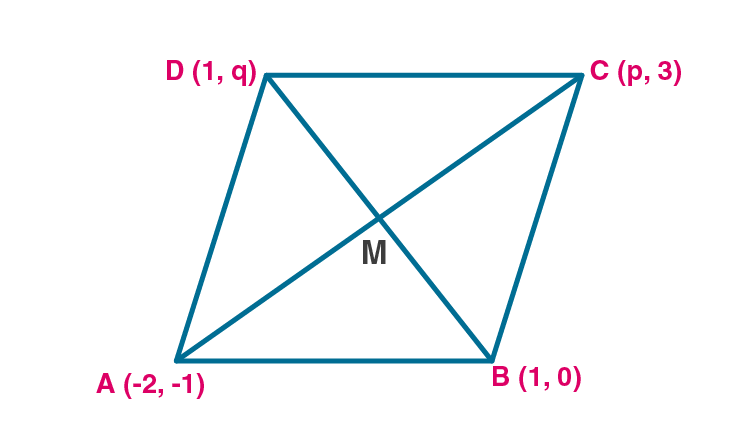Given vertices of the parallelogram are A(-2,-1), B(1, 0), C(p,3) and D(1,q).

Let M(x,y) be the midpoint of the diagonals of the parallelogram ABCD.

Diagonals AC and BD bisect each other at M.

When M is the midpoint of AC

By midpoint formula,

x = (-2+p)/2 = (p-2)/2 ..(i)

y = (-1+3)/2 = 2/2 = 1 ..(ii)

When M is the midpoint of BD

By midpoint formula,

x = (1+1)/2 = 2/2 = 1 ..(iii)

y = (q+0)/2 = q/2 ..(iv)

Equating (i) and (iii), we get

(p-2)/2 = 1

p-2 = 2

p = 2+2 = 4

Equating (i2) and (iv), we get

q/2 = 1

q = 2

Hence the value of p and q are 4 and 2 respectively.

25. If two vertices of a parallelogram are (3, 2) ( – 1, 0) and its diagonals meet at (2, – 5), find the other two vertices of the parallelogram.

Solution:

Let A(3,2) and B(-1,0) be the two vertices of the parallelogram ABCD.

Let M(2,-5) be the point where diagonals meet.

Since the diagonals of the parallelogram bisect each other, M is the midpoint of AC and BD.

Consider A-M-C

Let co-ordinate of C be (x2,y2)

x1 = 3, y1 = 2

x = 2, y = -5

By midpoint formula, x = (x1+x2)/2

2 = (3+x2)/2

3+x2 = 4

x2 = 4-3 = 1

By midpoint formula, y = (x1+x2)/2

-5 = (2+y2)/2

-10 = 2+y2

y2 = -10-2 = -12

Hence the co-ordinates of the point C are (1,-12).

Consider B-M-D

Let co-ordinate of D be (x2,y2)

x1 = -1, y1 = 0

x = 2, y = -5

By midpoint formula, x = (x1+x2)/2

2 = (-1+x2)/2

-1+x2 = 4

x2 = 4+1 = 5

By midpoint formula, y = (x1+x2)/2

-5 = (0+y2)/2

-10 = 0+y2

y2 = -10

Hence the co-ordinates of the point D are (5,-10).

26. Prove that the points A ( – 5, 4), B ( – 1, – 2) and C (5, 2) are the vertices of an isosceles right angled triangle. Find the co-ordinates of D so that ABCD is a square.

Solution: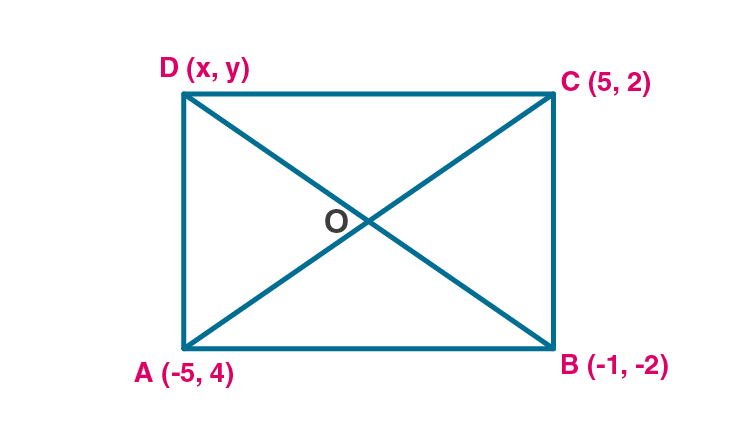Given points are A(-5,4), B(-1,-2) and C(5,2) are given.

Since these are vertices of an isosceles triangle ABC then AB = BC.

By distance formula, d(AB) = √[(x2-x1)2+(y2-y1)2]

Here x1 = -5, y1 = 4

x2 = -1, y2 = -2

d(AB) = √[(-1-(-5))2+(-2-4)2]

d(AB) = √[(4)2+(6)2]

d(AB) = √(16+36)

d(AB) = √52 …(i)

By distance formula, d(BC) = √[(x2-x1)2+(y2-y1)2]

Here x1 = -1, y1 = -2

x2 = 5, y2 = 2

d(BC) = √[(5-(-1))2+(2-(-2))2]

d(BC) = √[(6)2+(4)2]

d(BC) = √(36+16)

d(BC) = √52 …(ii)

From (i) and (ii) AB = BC

So given points are the vertices of isosceles triangle.

By distance formula, d(AC) = √[(x2-x1)2+(y2-y1)2]

Here x1 = -5, y1 = 4

x2 = 5, y2 = 2

d(AC) = √[(5-(-5))2+(2-4)2]

d(AC) = √[(10)2+(-2)2]

d(AC) = √(100+4)

d(AC) = √104 ….(iii)

Apply Pythagoras theorem to triangle ABC

AB2+BC2 = (√52)2+(√52)2

= 52+52

= 104 ….(iv)

AC2 = (√104)2 = 104…(v)

From (iv) and (v) we got

AB2+BC2 = AC2

So Pythagoras theorem is satisfied.

So the triangle is an isosceles right angled triangle.

Hence proved.

If ABCD is a square, let the diagonals meet at O.

Diagonals of a square bisect each other. So, O is the midpoint of AC and BD.

Consider A-O-C

x1 = -5, y1 = 4

x2 = 5, y2 = 2

By midpoint formula, x = (x1+x2)/2

x = (-5+5)/2 = 0/2 = 0

By midpoint formula, y = (y1+y2)/2

y = (4+2)/2 = 6/2 = 3

So co-ordinate of O is (0,3).

Consider B-O-D

Let co-ordinate of D be (x2,y2)

x1 = -1, y1 = -2

x = 0, y = 3

By midpoint formula, x = (x1+x2)/2

0 = (-1+x2)/2

-1+x2 = 0

x2 = 1

By midpoint formula, y = (x1+x2)/2

3 = (-2+y2)/2

6 = -2+y2

y2 = 6+2 = 8

Hence the co-ordinates of the point D are (1,8).

27. Find the third vertex of a triangle if its two vertices are ( – 1, 4) and (5, 2) and midpoint of one sides is (0, 3).

Solution: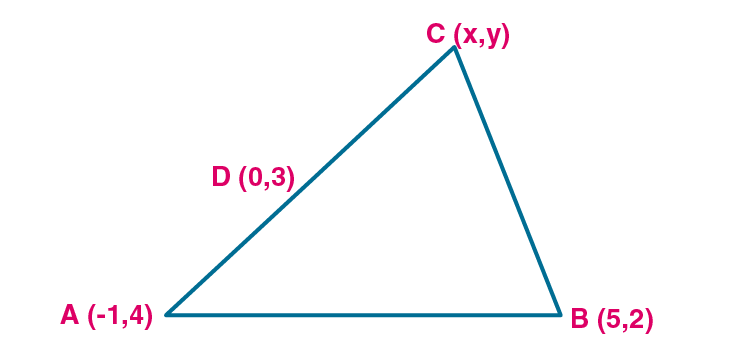Let A (-1,4) and B(5,2) are the vertices of the triangle and let D(0,3) is the midpoint of side AC.

Let co-ordinate of C be (x,y).

Consider D(0,3) as midpoint of AC

By midpoint formula,

(-1+x)/2 = 0

-1 +x = 0

x = 1

By midpoint formula,

(4+y)/2 = 3

4+y = 6

y = 6-4 = 2

So the co-ordinates of C are (1,2).

Consider D(0,3) as midpoint of BC

By midpoint formula,

(5+x)/2 = 0

5 +x = 0

x = -5

By midpoint formula,

(2+y)/2 = 3

2+y = 6

y = 6-2 = 4

So the co-ordinates of C are (-5,4).

Hence the co-ordinates of the point C will be (1,2) or (-5,4).

28. Find the coordinates of the vertices of the triangle the middle points of whose sides are (0, ½ ) , ( ½ , ½) and ( ½ , 0).

Solution: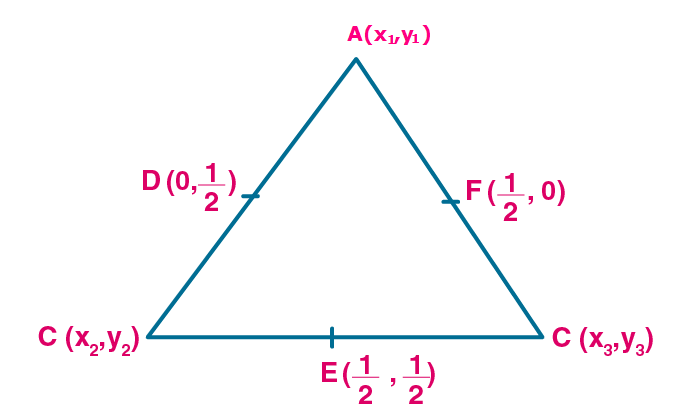Let A(x1,y1), B(x2,y2) and C(x3,y3) be the vertices of the triangle ABC.

Consider AB

By midpoint formula, (x1+x2)/2 = 0

x1+x2 = 0

x1 = -x2 ..(i)

By midpoint formula, (y1+y2)/2 = ½

y1+y2 = 1 …(ii)

Consider AC

By midpoint formula, (x1+x3)/2 = ½

x1+x3 = 1 …(iii)

By midpoint formula, (y1+y3)/2 = 0

y1+y3 = 0

y1 = -y3 …(iv)

Consider BC

By midpoint formula, (x2+x3)/2 = ½

x2+x3 = 1 …(v)

By midpoint formula, (y2+y3)/2 = ½

y2+y3 = 1 …(vi)

Substitute (i) in (iii)

Then (iii) becomes -x2+x3 = 1

Equation (v) x2+x3 = 1

Adding above two equations, we get

2x3 = 2

x3 = 2/2 = 1

Substitute x3 = 1 in (iii), we get x1 = 0

x2 = 0 [From (i)]

So x1 = 0, x2 = 0, x3 = 1

Substitute (iv) in (ii)

Then (ii) becomes -y3+y2 = 1

Equation (vi) y2+y3 = 1

Adding above two equations, we get

2y2 = 2

y2 = 2/2 = 1

Substitute y2 = 1 in (i), we get y1 = 0

y3 = 0

So y1 = 0, y2 = 1, y3 = 0

Hence the Co-ordinates of vertices are A(0,0), B(0,1) and C(1,0).

29. Show by section formula that the points (3, – 2), (5, 2) and (8, 8) are collinear.

Solution:

Let the point B(5,2) divides the line joining A(3,-2) and C(8,8) in the ratio m:n.

Then by section formula, x = (mx2+nx1)/(m+n)

5 = (m×8+n×3)/(m+n)

5 = (8m+3n)/(m+n)

5m+5n = 8m+3n

2n = 3m

m/n = 2/3 …(i)

By section formula, y = (my2+ny1)/(m+n)

2 = (m×8+n×-2)/(m+n)

2 = (8m-2n)/(m+n)

2m+2n = 8m-2n

6m = 4n

m/n = 4/6 = 2/3 …(ii)

Here ratios are same.

So the points are collinear.

30. Find the value of p for which the points ( – 5, 1), (1, p) and (4, – 2) are collinear.

Solution:

Let A(-5,1) divides the line joining (1,p) and (4,-2) in the ratio m:n

Then by section formula, x = (mx2+nx1)/(m+n)

-5 = (m×4+n×1)/(m+n)

-5 = (4m+n)/(m+n)

-5m-5n = 4m+n

-9m = 6n

m/n = -9/6 = -2/3 …(i)

By section formula, y = (my2+ny1)/(m+n)

1 = (m×-2+n×p)/(m+n)

1 = (-2m+pn)/(m+n)

m+n = -2m+pn

3m = (p-1)n

m/n = (p-1)/3 ….(ii)

Equating (i) and (ii)

(p-1)/3 = -2/3

p-1 = -2

p = -2+1 = -1

Hence the value of p is -1.

31. A (10, 5), B (6, – 3) and C (2, 1) are the vertices of triangle ABC. L is the mid point of AB, M is the mid-point of AC. Write down the co-ordinates of L and M. Show that LM = ½ BC.

Solution:

Given points are A(10,5), B(6,-3) and C(2,1).

Let L(x,y) be the midpoint of AB.

Here x1= 10, y1 = 5

x2 = 6, y2 = -3

By midpoint formula, x = (x1+x2)/2

x = (10+6)/2 = 16/2 = 8

By midpoint formula, y = (y1+y2)/2

y = (5-3)/2 = 2/2 = 1

So co-ordinates of L are (8,1).

Let M(x,y) be the midpoint of AC.

Here x1= 10, y1 = 5

x2 = 2, y2 = 1

By midpoint formula, x = (x1+x2)/2

x = (10+2)/2 = 12/2 = 6

By midpoint formula, y = (y1+y2)/2

y = (5+1)/2 = 6/2 = 3

So co-ordinates of M are (6,3).

By distance formula, d(LM) = √[(x2-x1)2+(y2-y1)2]

The points are L(8,1) and M(6,3)

So x1= 8, y1 = 1

x2 = 6, y2 = 3

d(LM) = √[(x2-x1)2+(y2-y1)2]

d(LM) = √[(6-8)2+(3-1)2]

d(LM) = √[(-2)2+(2)2]

d(LM) = √(4+4)

d(LM) = √8 = 2√2 …(i)

By distance formula, d(BC) = √[(x2-x1)2+(y2-y1)2]

The points are B(6,-3) and C(2,1).

So x1= 6, y1 = -3

x2 = 2, y2 = 1

d(BC) = √[(x2-x1)2+(y2-y1)2]

d(BC) = √[(2-6)2+(1-(-3))2]

d(BC) = √[(-4)2+(4)2]

d(BC) = √(16+16)

d(BC) = √32 = 4√2 …(ii)

From (i) and (ii), LM = ½ BC

32. A (2, 5), B ( – 1, 2) and C (5, 8) are the vertices of a triangle ABC. P and Q are points on AB and AC respectively such that AP : PB = AQ : QC = 1 : 2.

(i) Find the co-ordinates of P and Q.

(ii) Show that PQ = 1/3 BC

Solution: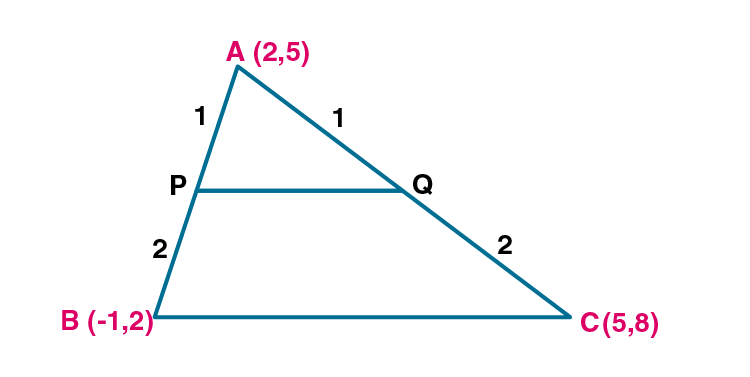(i) Given vertices of the ABC are A(2,5), B(-1,2) and C(5,8).

P and Q are points on AB and AC respectively such that AP:PB = AQ :QC = 1:2.

P(x,y) divides AB in the ratio 1:2.

x1= 2, y1 = 5

x2 = -1, y2 = 2

m:n = 1:2

By section formula, x = (mx2+nx1)/(m+n)

x = (1×-1+2×2)/(1+2)

x = (-1+4)/(3)

x = 3/3 = 1

By section formula, y = (my2+ny1)/(m+n)

y = (1×2+2×5)/(1+2)

y = (2+10)/(3)

y = 12/3 = 4

Co-ordinates of P are (1,4).

Q(x,y) divides AC in the ratio 1:2.

x1= 2, y1 = 5

x2 = 5, y2 = 8

m:n = 1:2

By section formula, x = (mx2+nx1)/(m+n)

x = (1×5+2×2)/(1+2)

x = (5+4)/(3)

x = 9/3 = 3

By section formula, y = (my2+ny1)/(m+n)

y = (1×8+2×5)/(1+2)

y = (8+10)/(3)

y = 18/3 = 6

Co-ordinates of Q are (3,6).

(ii) By distance formula, d(PQ) = √[(x2-x1)2+(y2-y1)2]

Points are P(1,4) and Q(3,6).

So x1= 1, y1 = 4

x2 = 3, y2 = 6

d(PQ) = √[(x2-x1)2+(y2-y1)2]

d(PQ) = √[(3-1)2+(6-4)2]

d(PQ) = √[(2)2+(2)2]

d(PQ) = √(4+4)

d(PQ) = √8 = 2√2 ..(i)

By distance formula, d(BC) = √[(x2-x1)2+(y2-y1)2]

Points are B(-1,2) and C(5,8).

So x1= -1, y1 = 2

x2 = 5, y2 = 8

d(BC) = √[(x2-x1)2+(y2-y1)2]

d(BC) = √[(5-(-1))2+(8-2)2]

d(BC) = √[(6)2+(6)2]

d(BC) = √(36+36)

d(BC) = √72 = √(36×2) = 6√2 ..(ii)

BC/3 = 6√2/3 = 2√2 = PQ

PQ = 1/3 BC.

Hence proved.

33. The mid-point of the line segment AB shown in the adjoining diagram is (4, – 3). Write down the co-ordinates of A and B.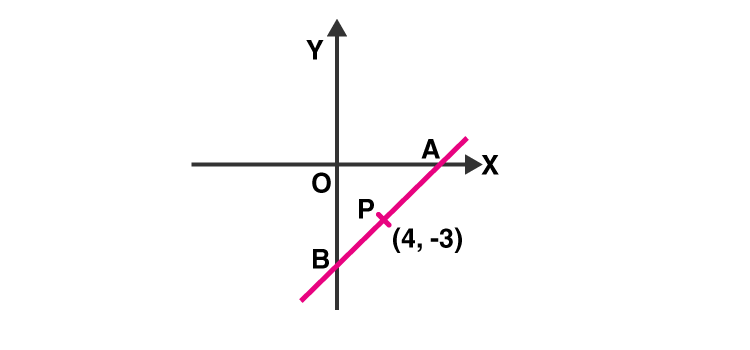Solution:

Let P(4,-3) be the midpoint of line joining the points A and B.

Since A lies on X axis, its co-ordinates are (x2,0)

Since B lies on Y axis, its co-ordinates are (0,y1)

By midpoint formula, x = (x1+x2)/2

4 = (0+x2)/2

x2 = 4×2 = 8

By midpoint formula, y = (y1+y2)/2

-3 = (y1+0)/2

y1 = -3×2 = -6

Hence the co-ordinates of A and B are (8,0)and (0,-6) respectively.

34. Find the co-ordinates of the centroid of a triangle whose vertices are A ( – 1, 3), B(1, – 1) and C (5, 1) (2006)

Solution:

Given vertices of the triangle are A(-1,3), B(1,-1) and C(5,1)

Co-ordinates of the centroid of a triangle, whose vertices are (x1,y1), (x2,y2) and (x3,y3) are

[(x1 + x2+ x3)/3, (y1 + y2+ y3)/3]

(x1,y1) = (-1,3)

(x2,y2) = (1,-1)

(x3,y3) = (5,1)

(x1 + x2+ x3)/3 = (-1+1+5)/3 = 5/3

(y1 + y2+ y3)/3 = (3-1+1)/3 = 3/3 = 1

Hence the co-ordinates of centroid are (5/3, 1).

35. Two vertices of a triangle are (3, – 5) and ( – 7, 4). Find the third vertex given that the centroid is (2, – 1).

Solution:
Let third vertex be C(x3,y3).

Given (x1,y1) = (3,-5)

(x2,y2) = (-7,4)

Co-ordinates of centroid are (2,-1)

Co-ordinates of the centroid of a triangle, whose vertices are (x1,y1), (x2,y2) and (x3,y3) are

[(x1 + x2+ x3)/3, (y1 + y2+ y3)/3]

(x1 + x2+ x3)/3 = (3+-7+x3)/3 = 2 [x co-ordinate of centroid]

-4+x3 = 2×3

-4+x3 = 6

x3 = 6+4

x3 = 10

(y1 + y2+ y3)/3 = -1 [y co-ordinate of centroid]

-5+4+y3 = -1×3

-1+y3 = -3

y3 = -3+1

y3 = -2

Hence the third vertex is (10,-2).

36. The vertices of a triangle are A ( – 5, 3), B (p, – 1) and C (6, q). Find the values of p and q if the centroid of the triangle ABC is the point (1, – 1).

Solution:

Given vertices of the triangle are A(-5,3), B(p,-1) and C(6,q).

Co-ordinates of centroid are (1,-1).

Co-ordinates of the centroid of a triangle, whose vertices are (x1,y1), (x2,y2) and (x3,y3) are

[(x1 + x2+ x3)/3, (y1 + y2+ y3)/3]

(x1,y1) = (-5,3)

(x2,y2) = (p,-1)

(x3,y3) = (6,q)

x co-ordinate of centroid, (x1 + x2+ x3)/3 = (-5+p+6)/3 = 1

p+1 = 3

p = 3-1

p = 2

y co-ordinate of centroid, (y1 + y2+ y3)/3 = (3-1+q)/3 = -1

2+q = 3×-1

2+q = -3

q = -3-2

q = -5

Hence the value of p and q are 2 and -5 respectively.